# RD Sharma Solutions for Class 10 Maths Chapter 9 Arithmetic Progressions Exercise 9.6

Arithmetic progressions is a high weightage chapter in Class 10 Maths. This exercise deals with problems related to the sum of terms of an A.P. Numerous real-time problems are discussed under this exercise and the RD Sharma Solutions Class 10 is the best tool for students to refer and prepare well for their exams. This exercise solutions of RD Sharma Solutions for Class 10 Maths Chapter 9 Arithmetic Progressions Exercise 9.6 PDF is given below.

## RD Sharma Solutions for Class 10 Maths Chapter 9 Arithmetic Progressions Exercise 9.6 Download PDF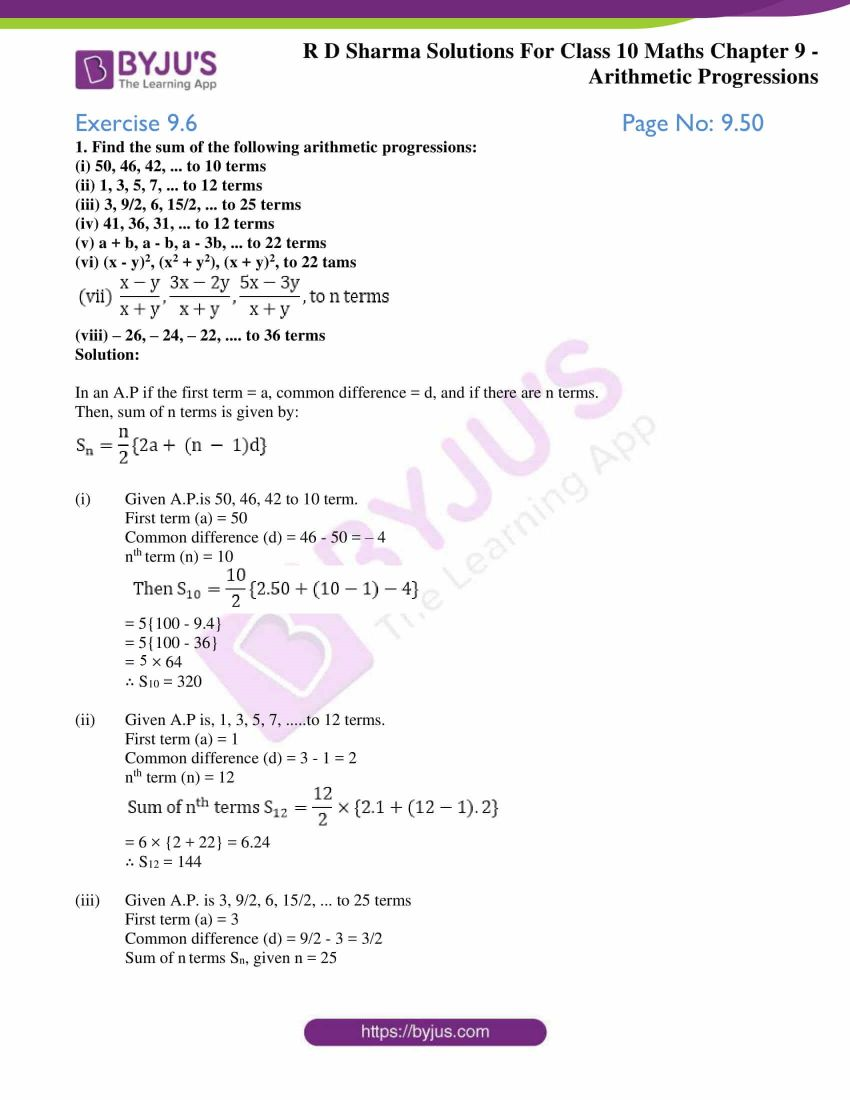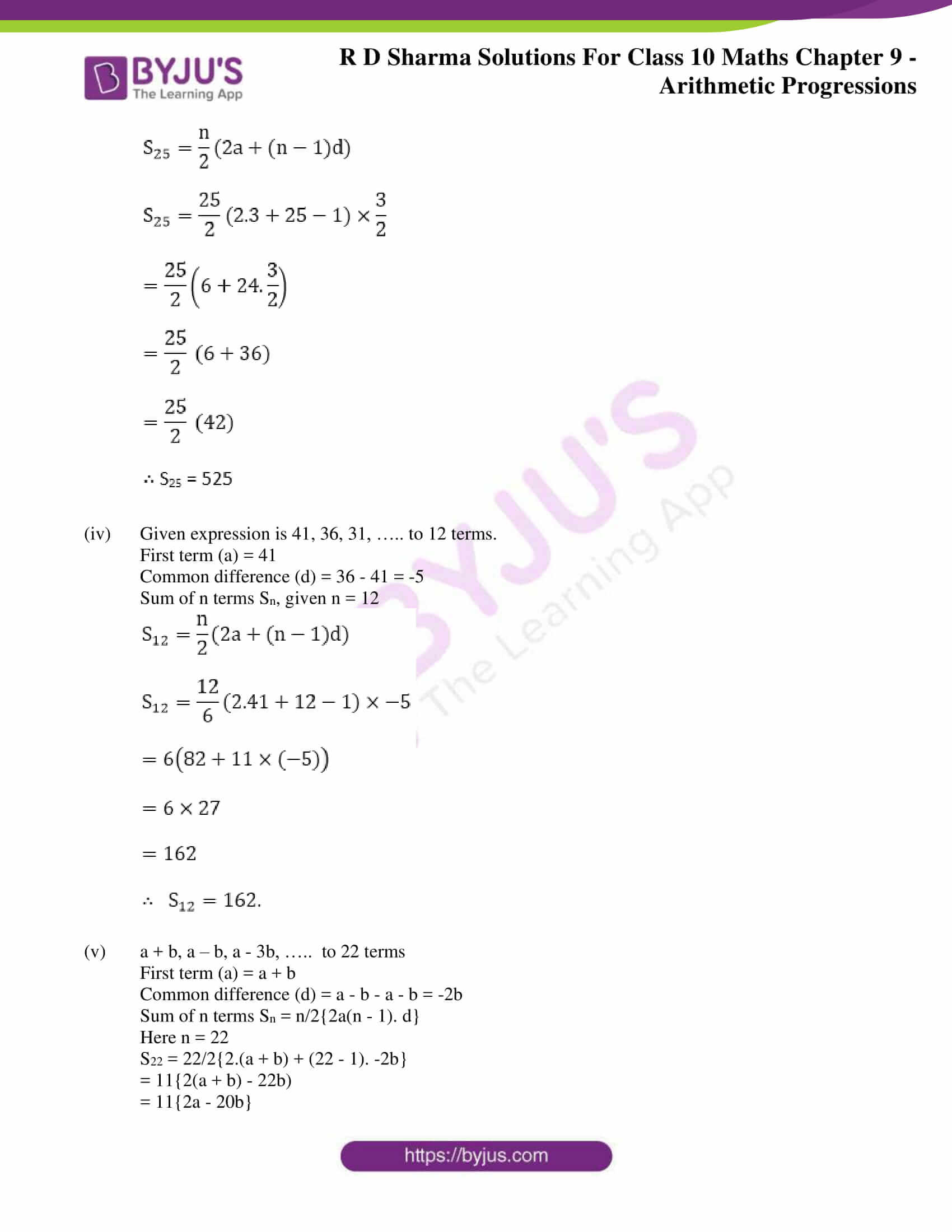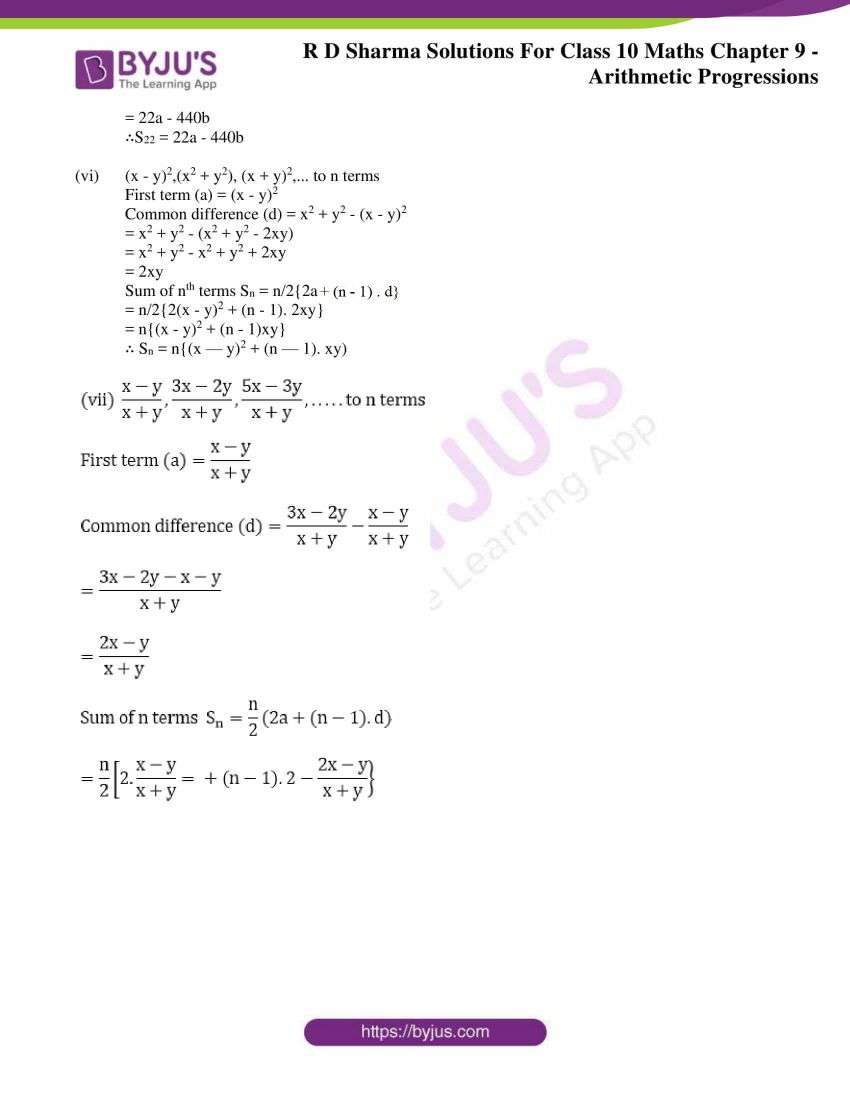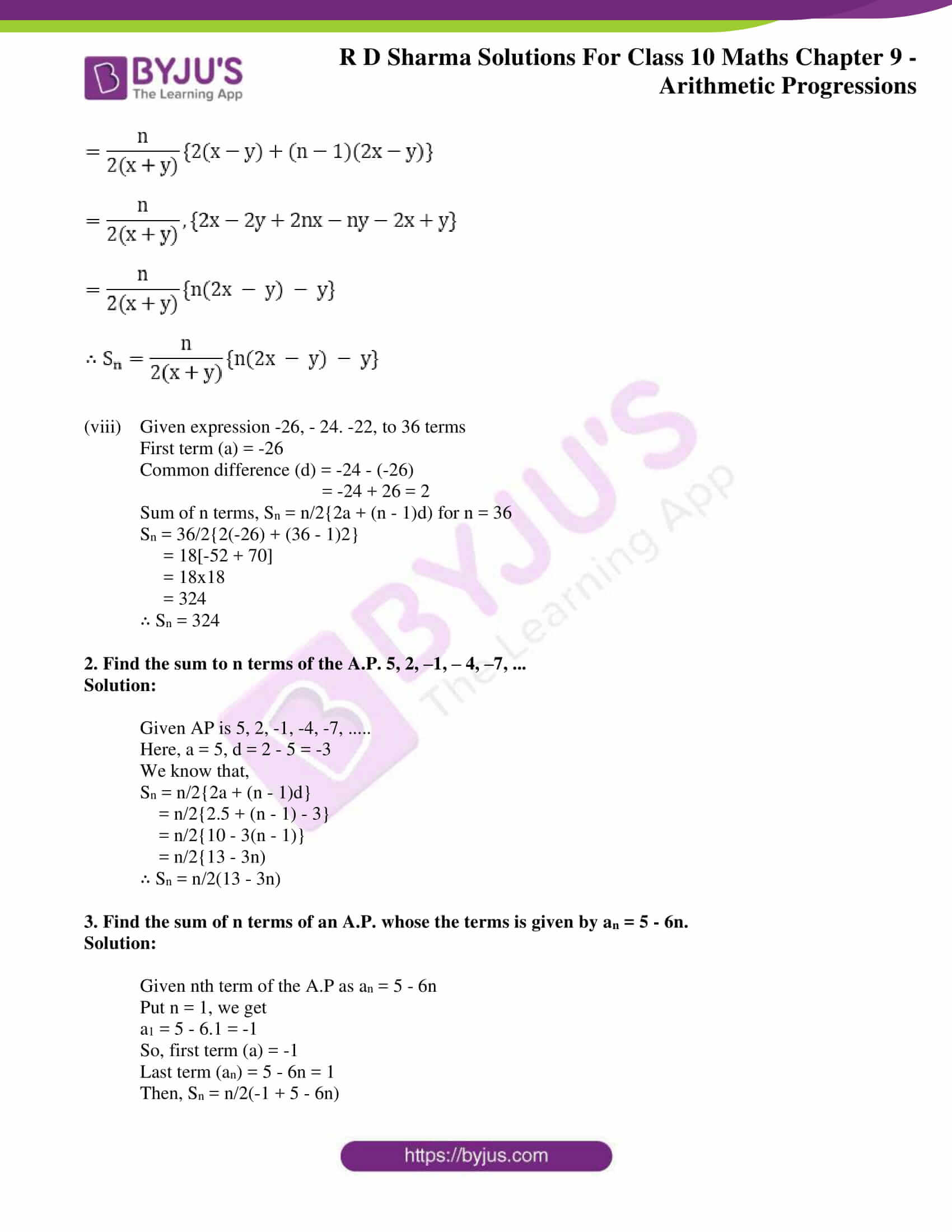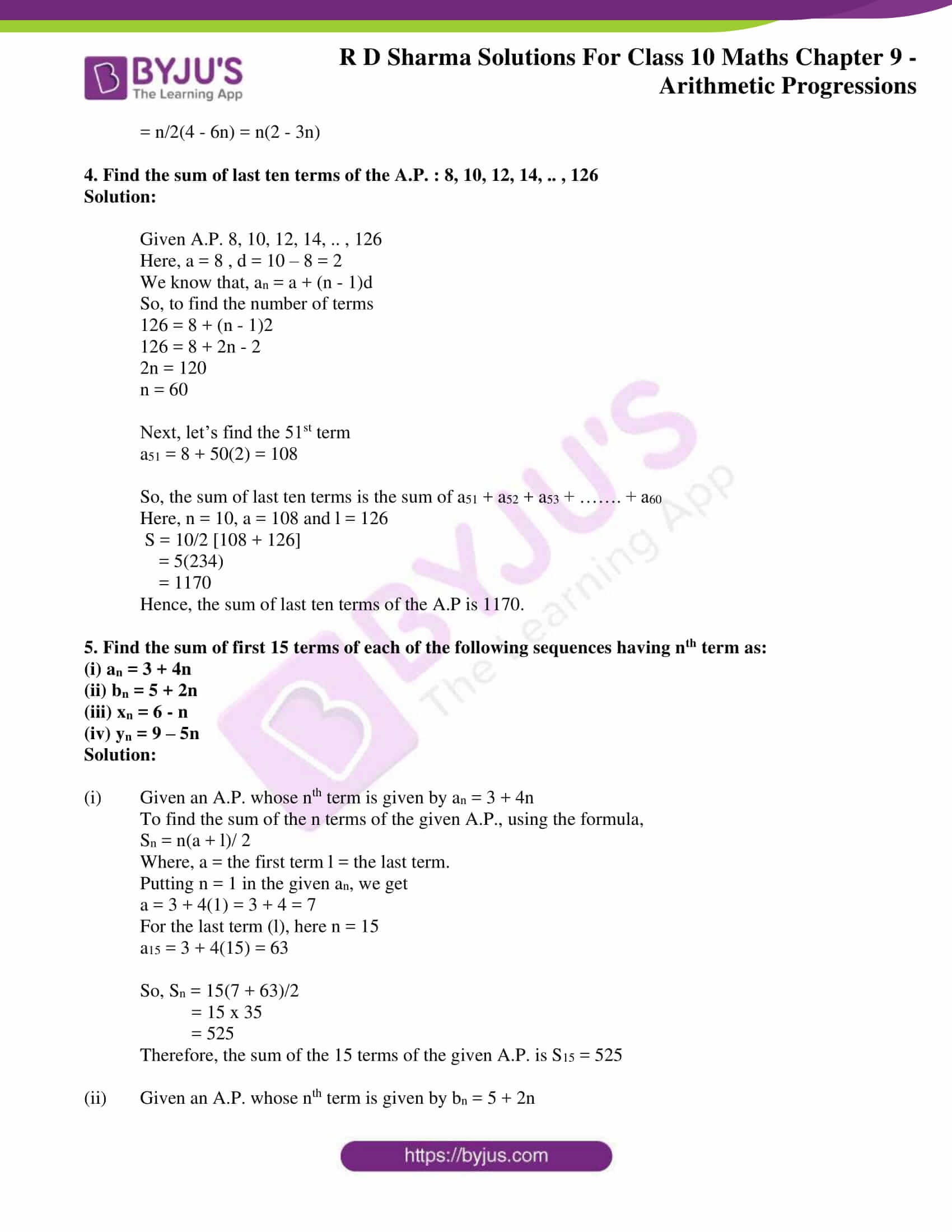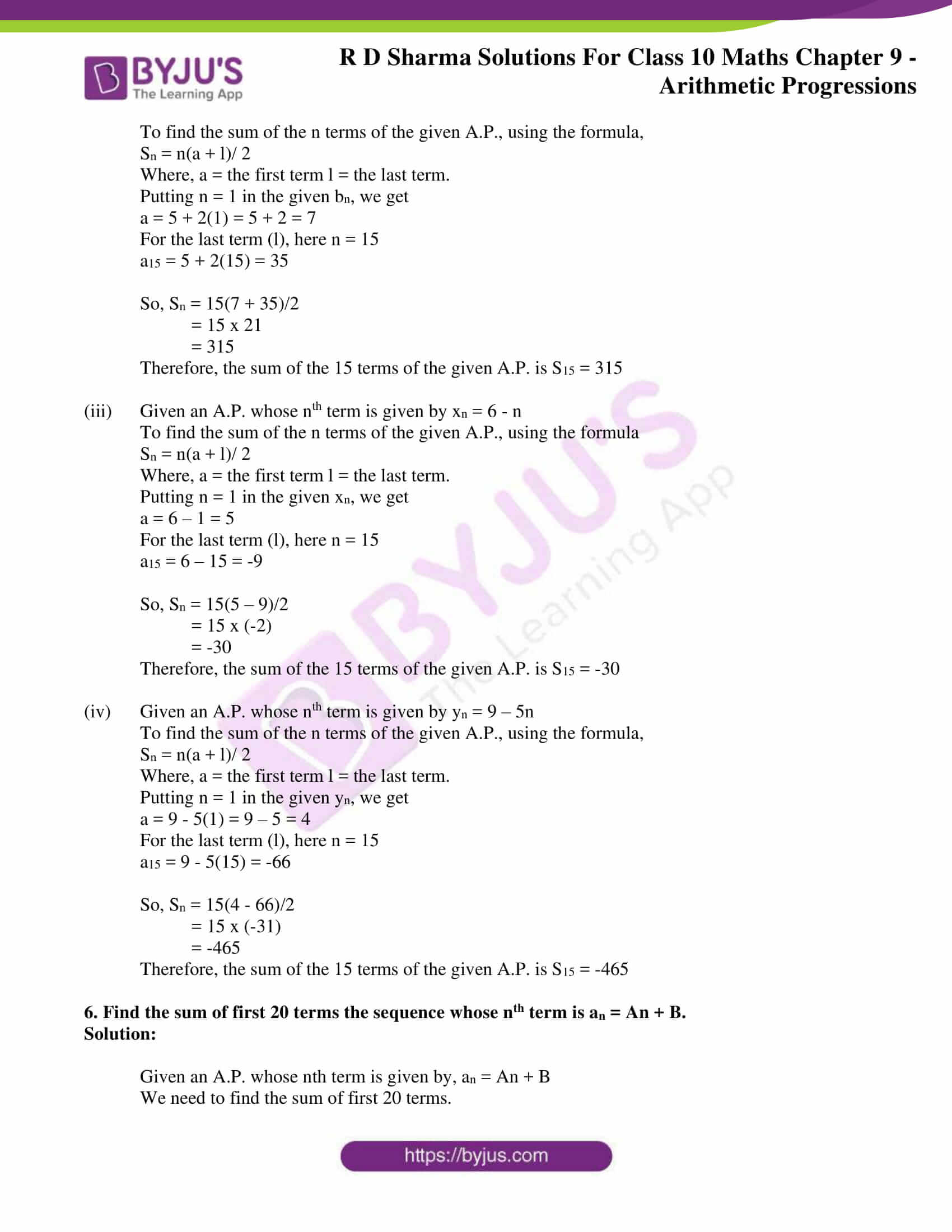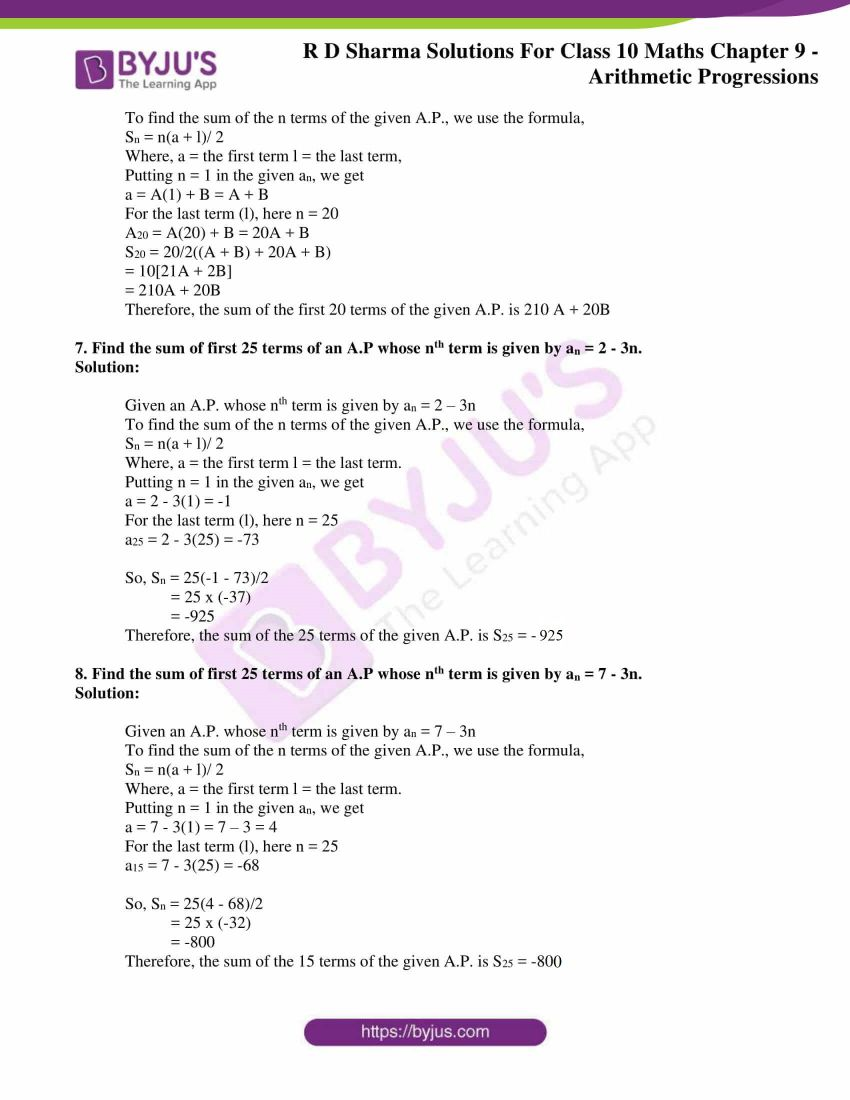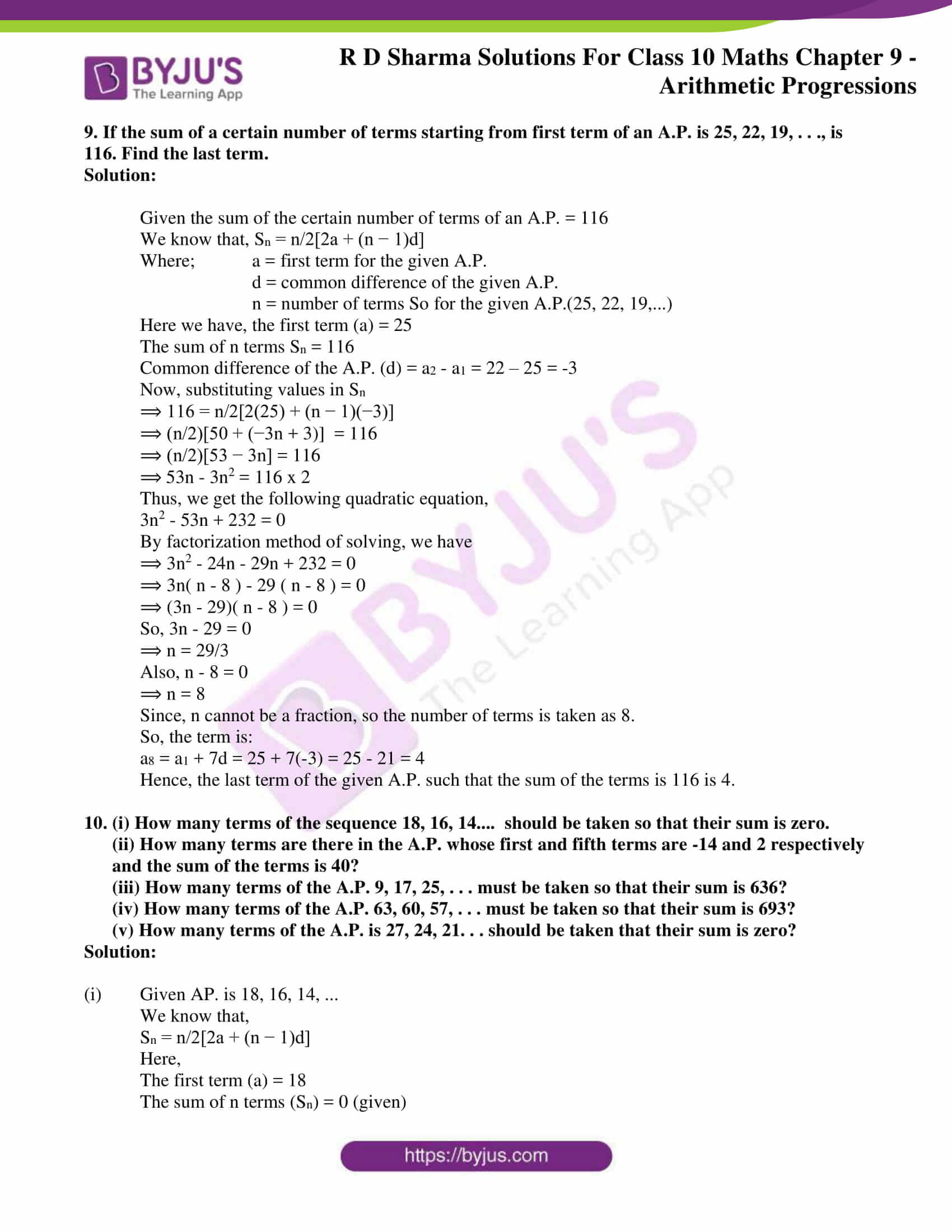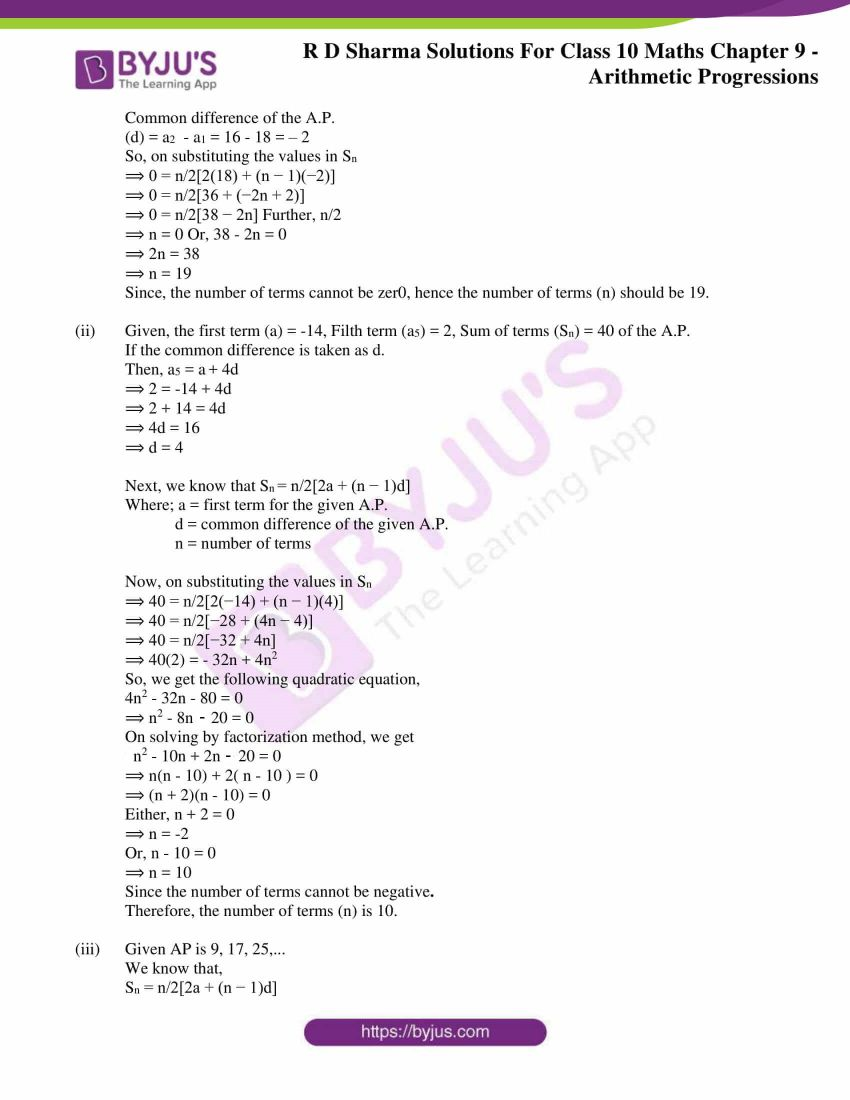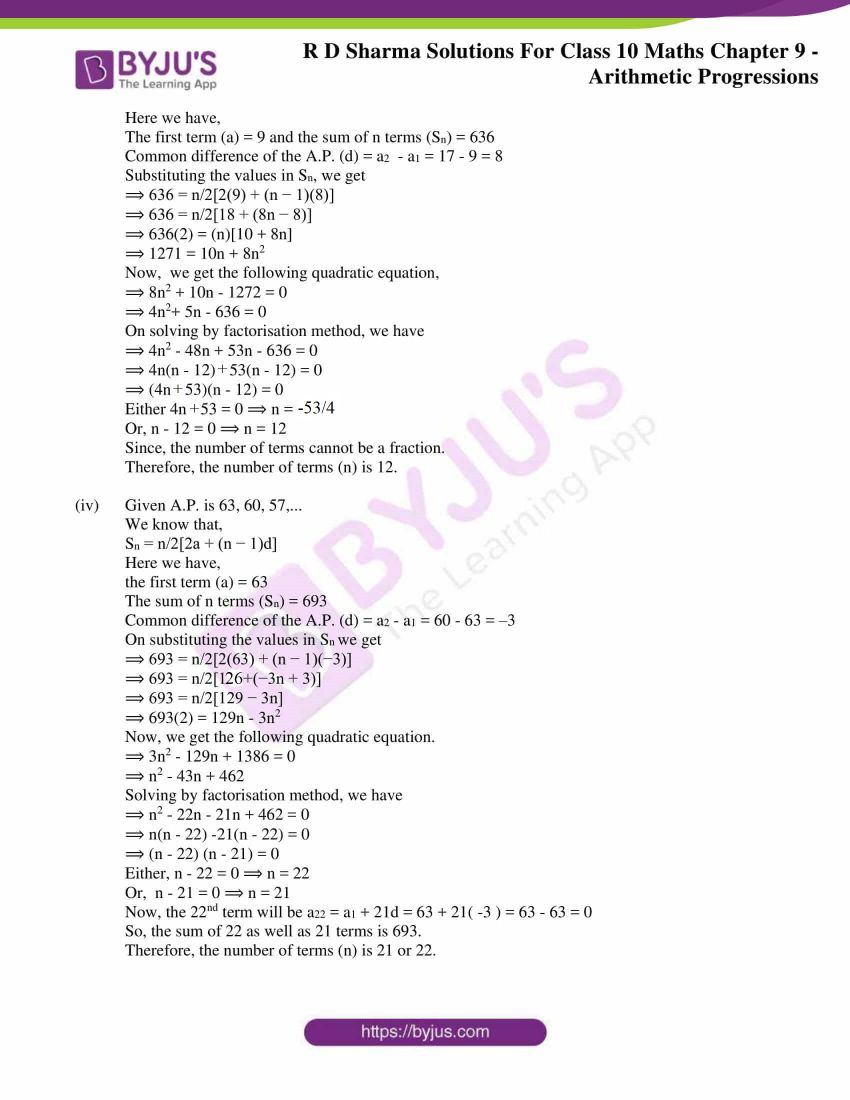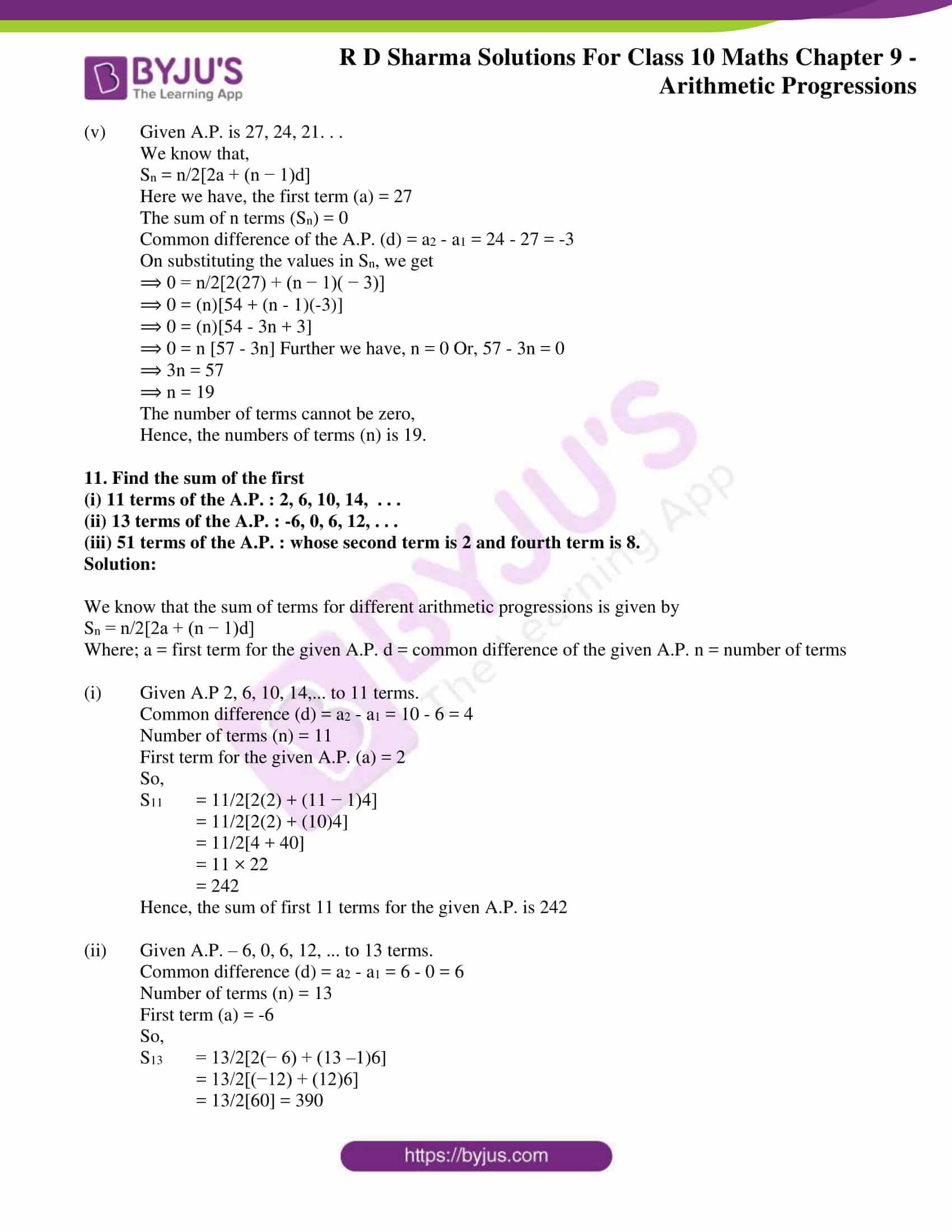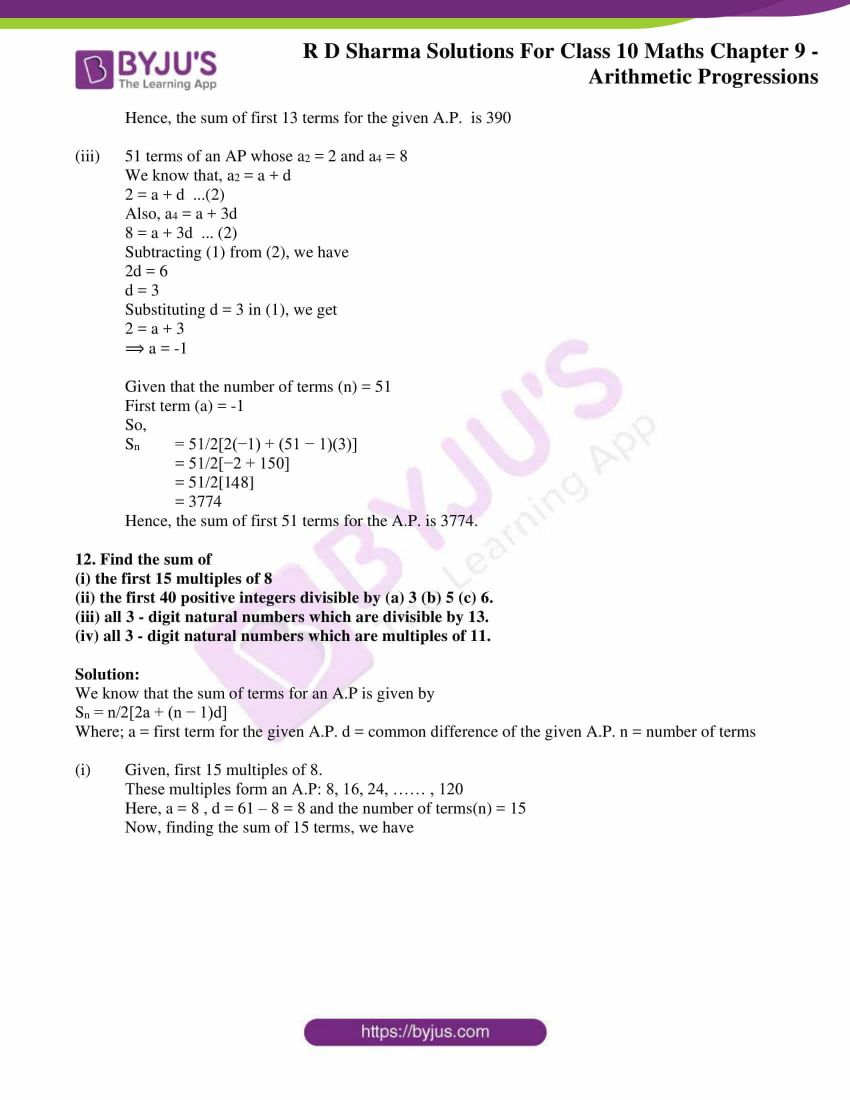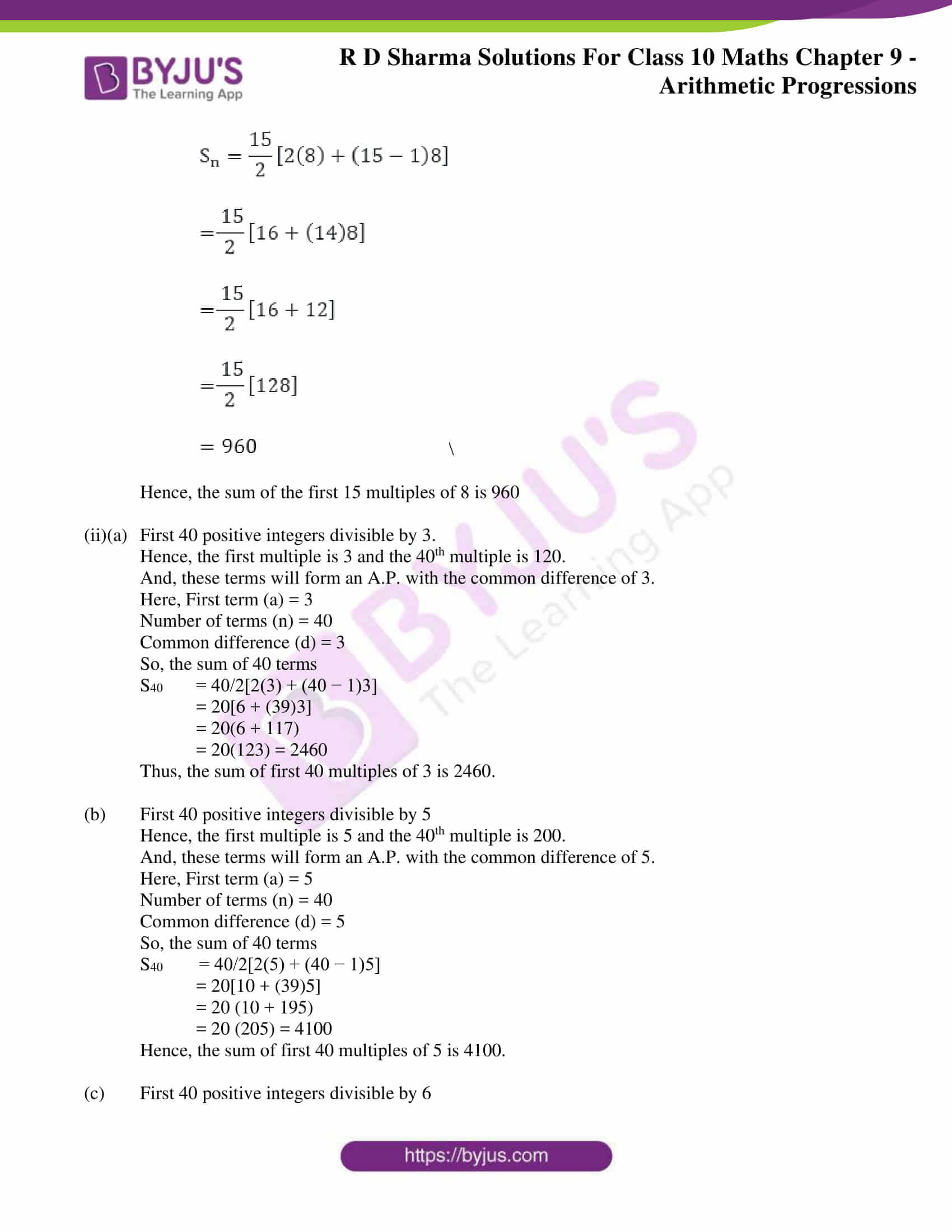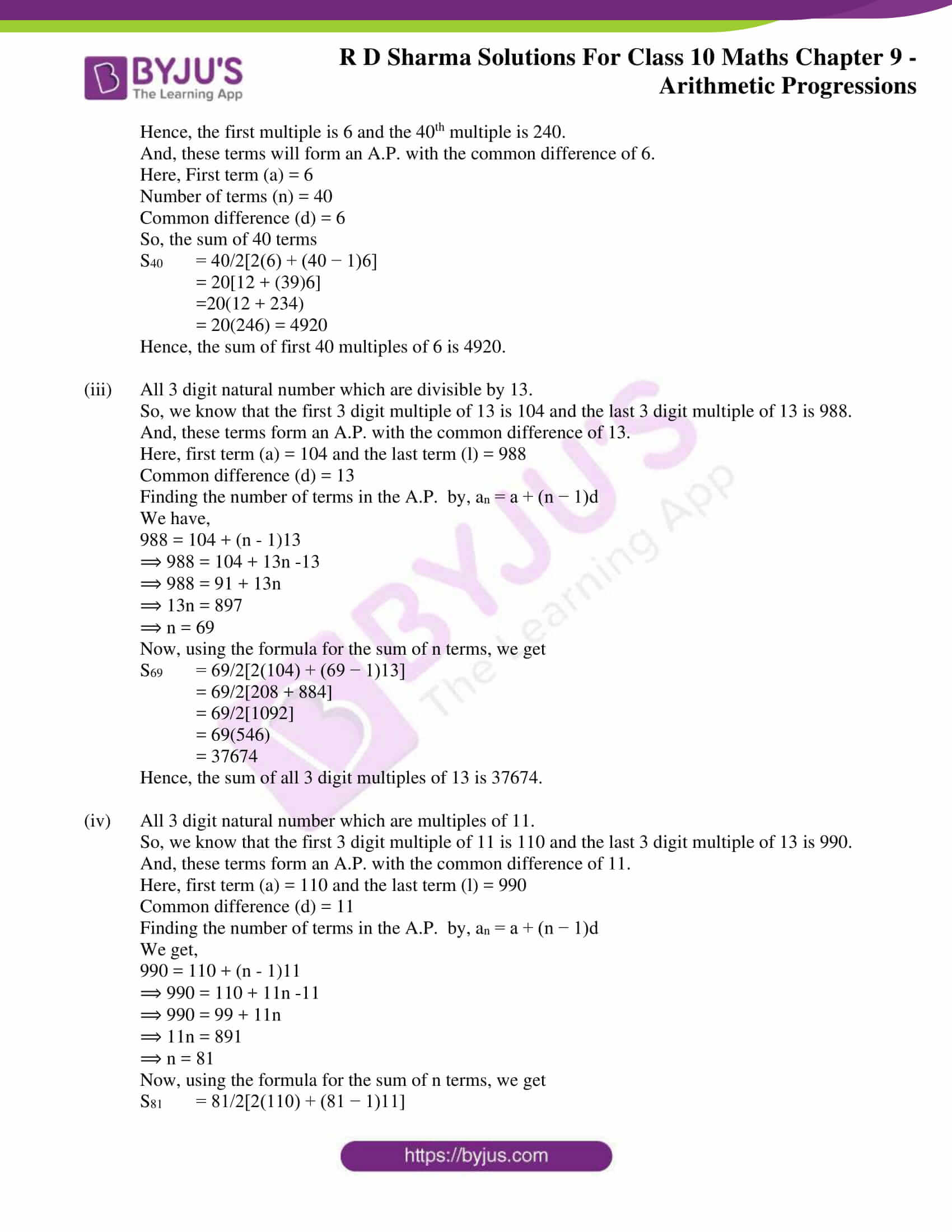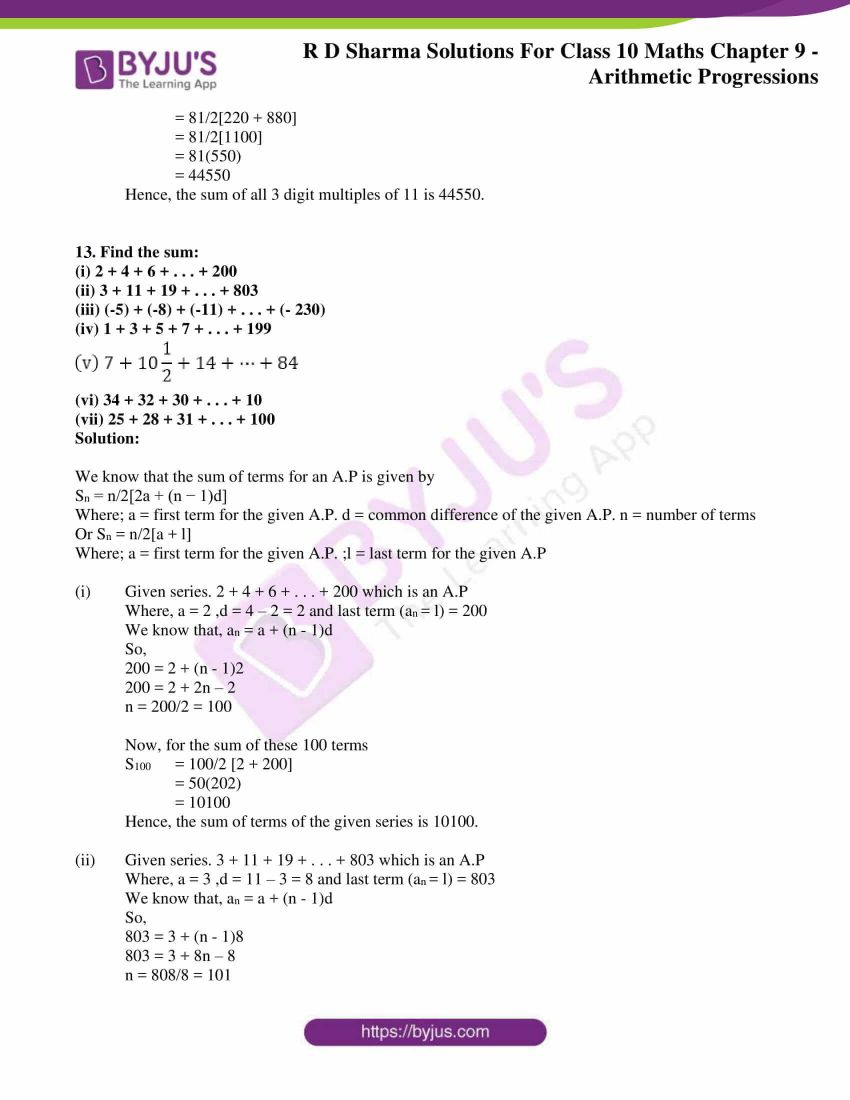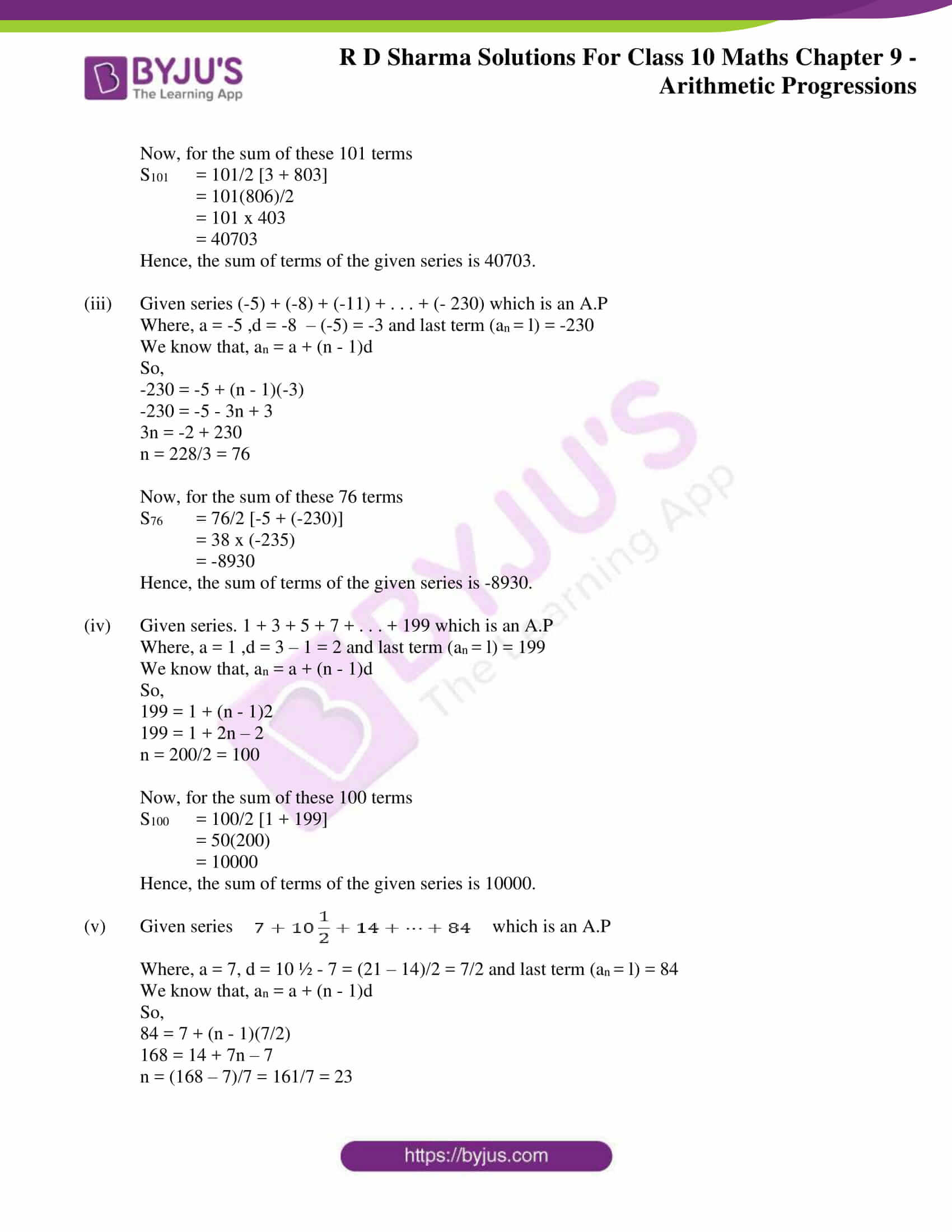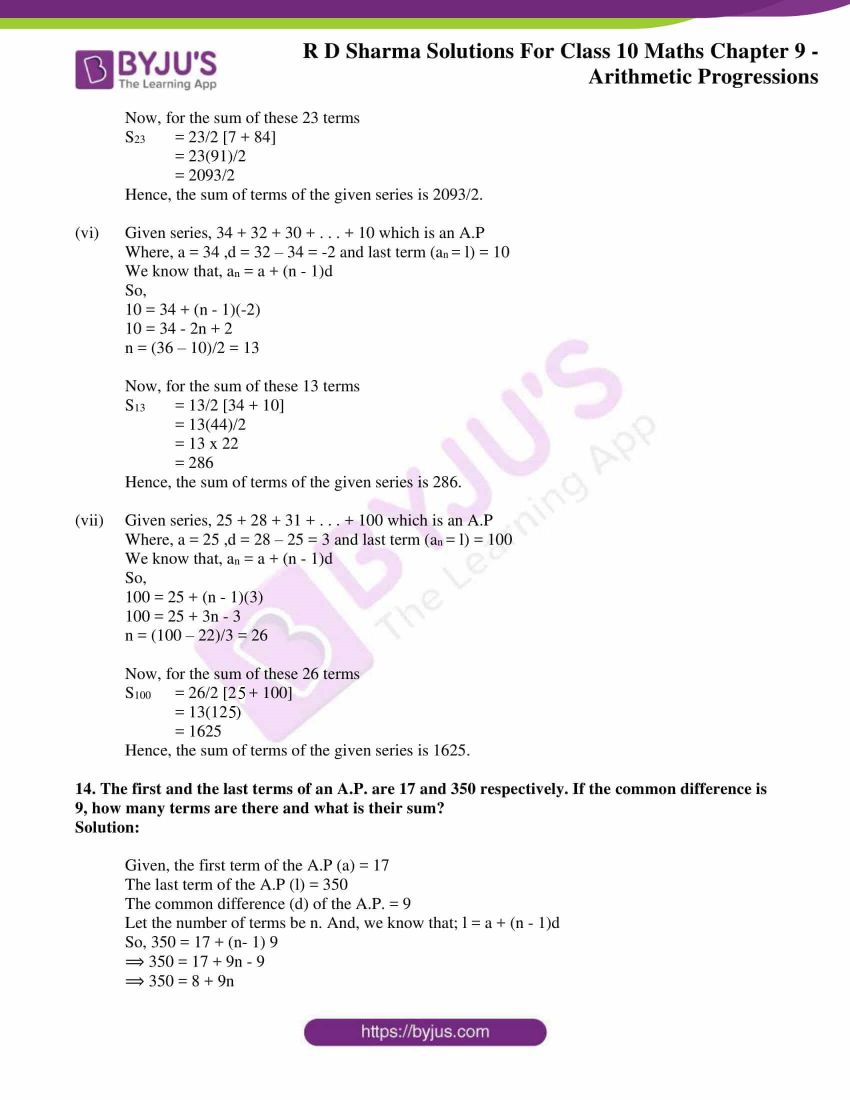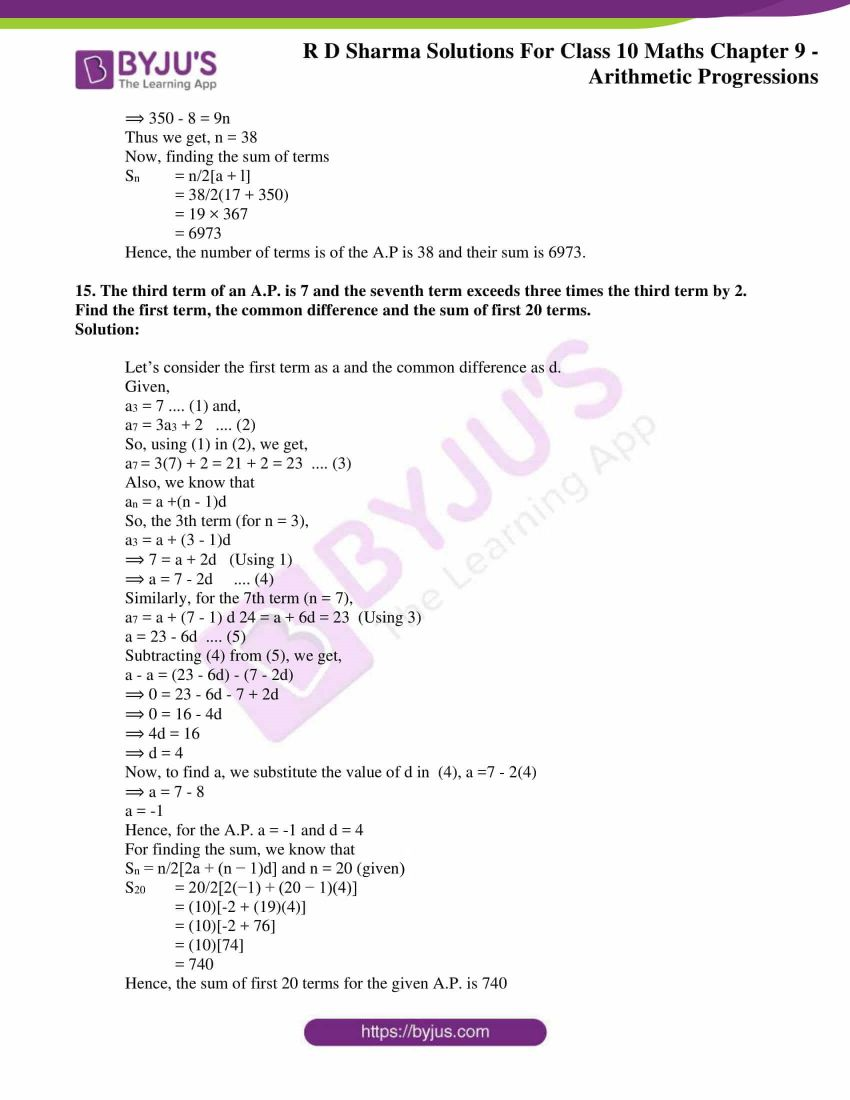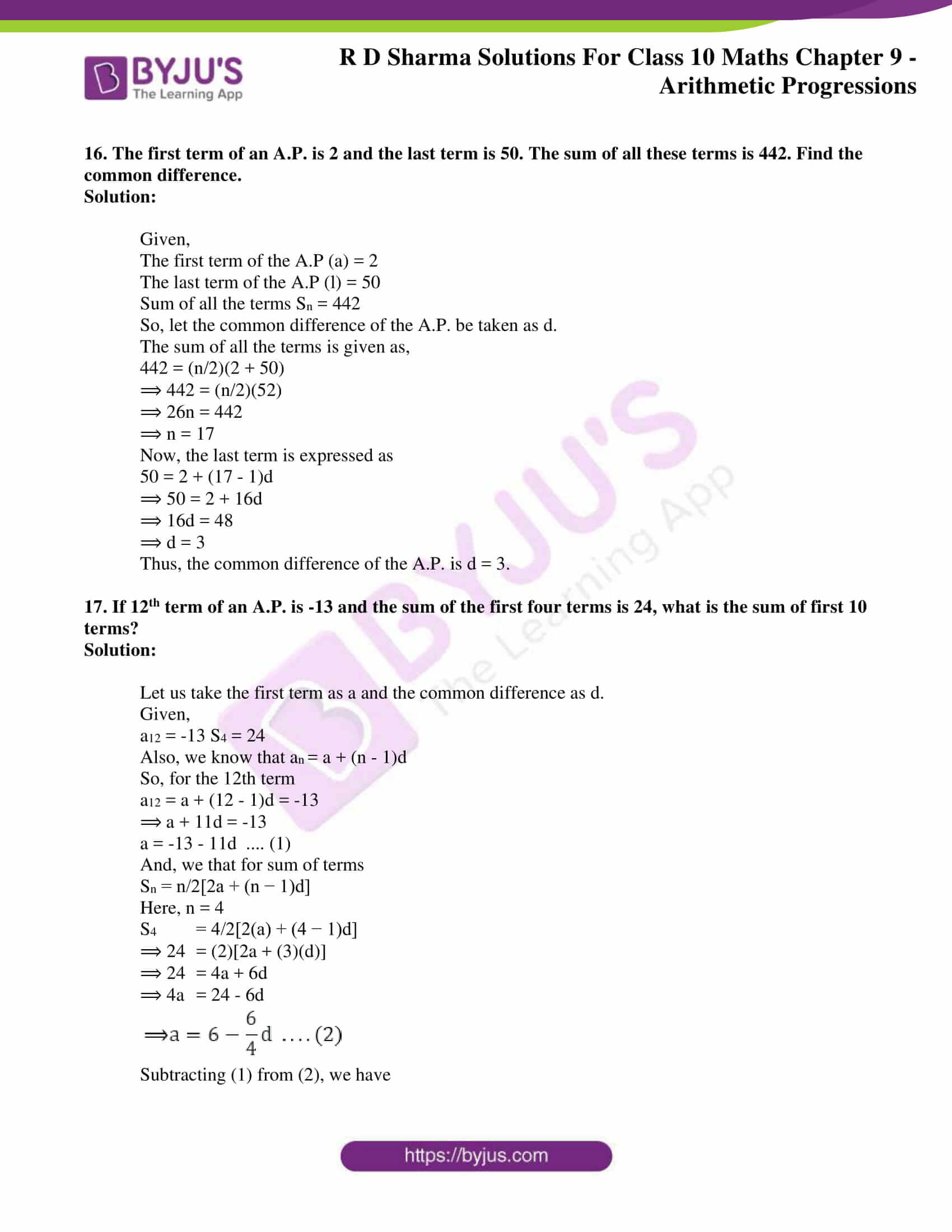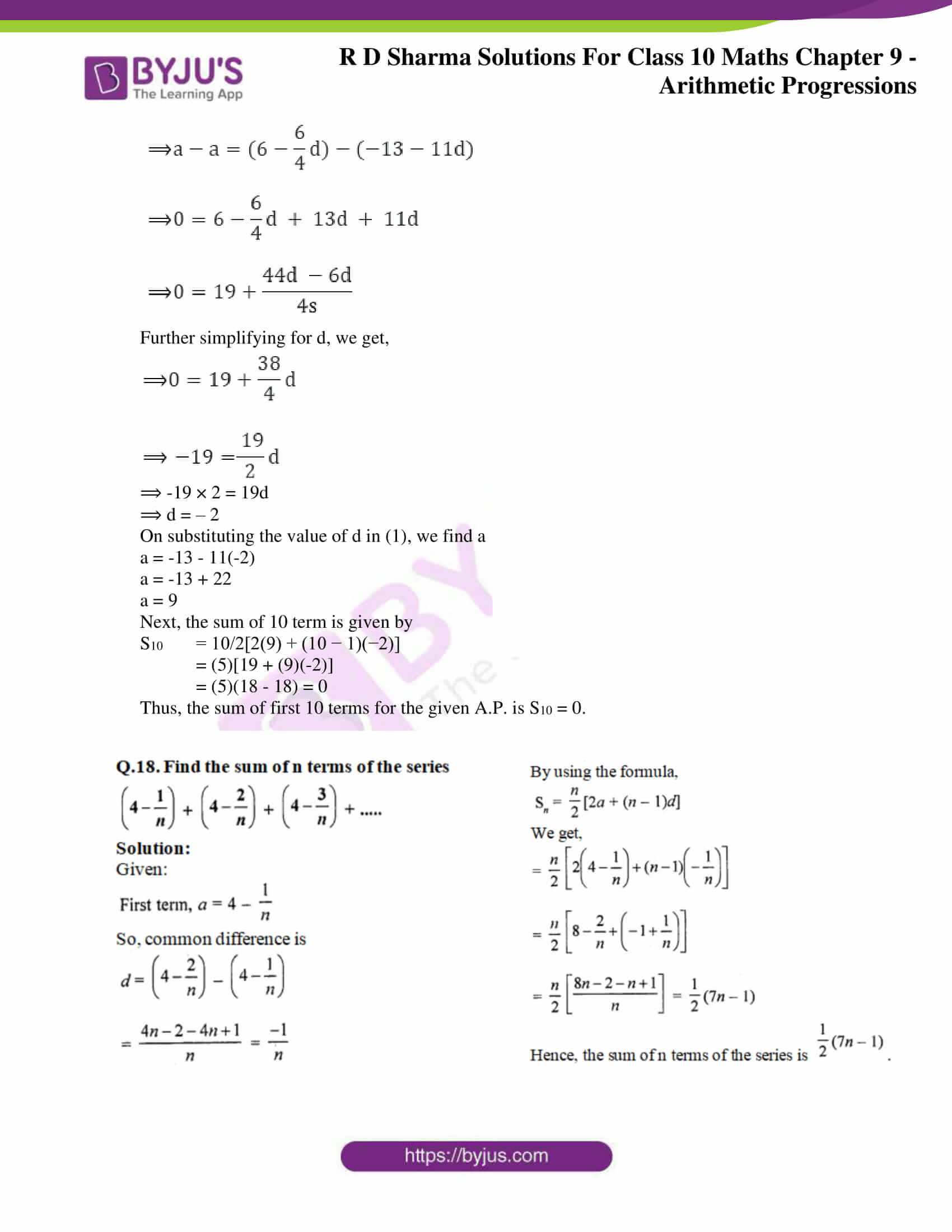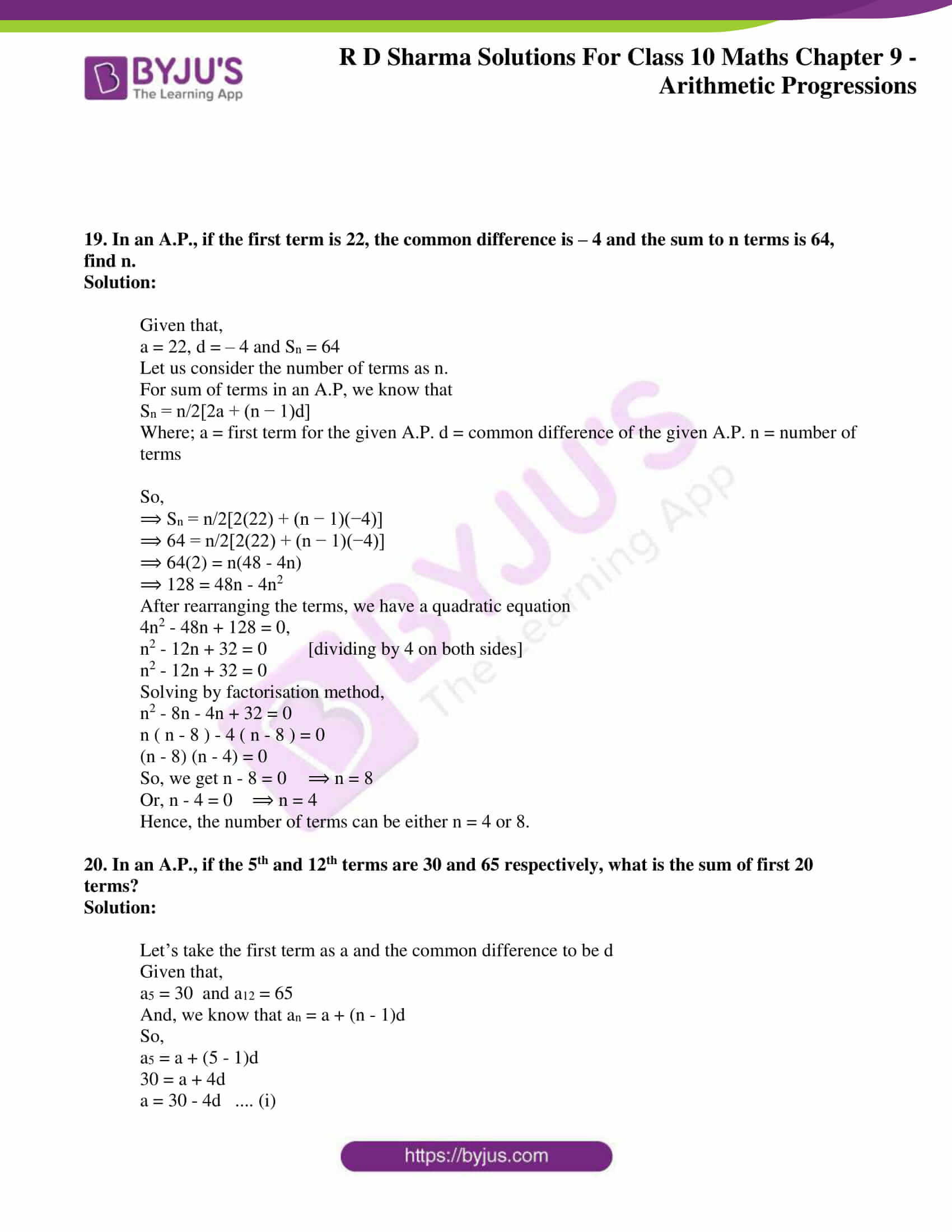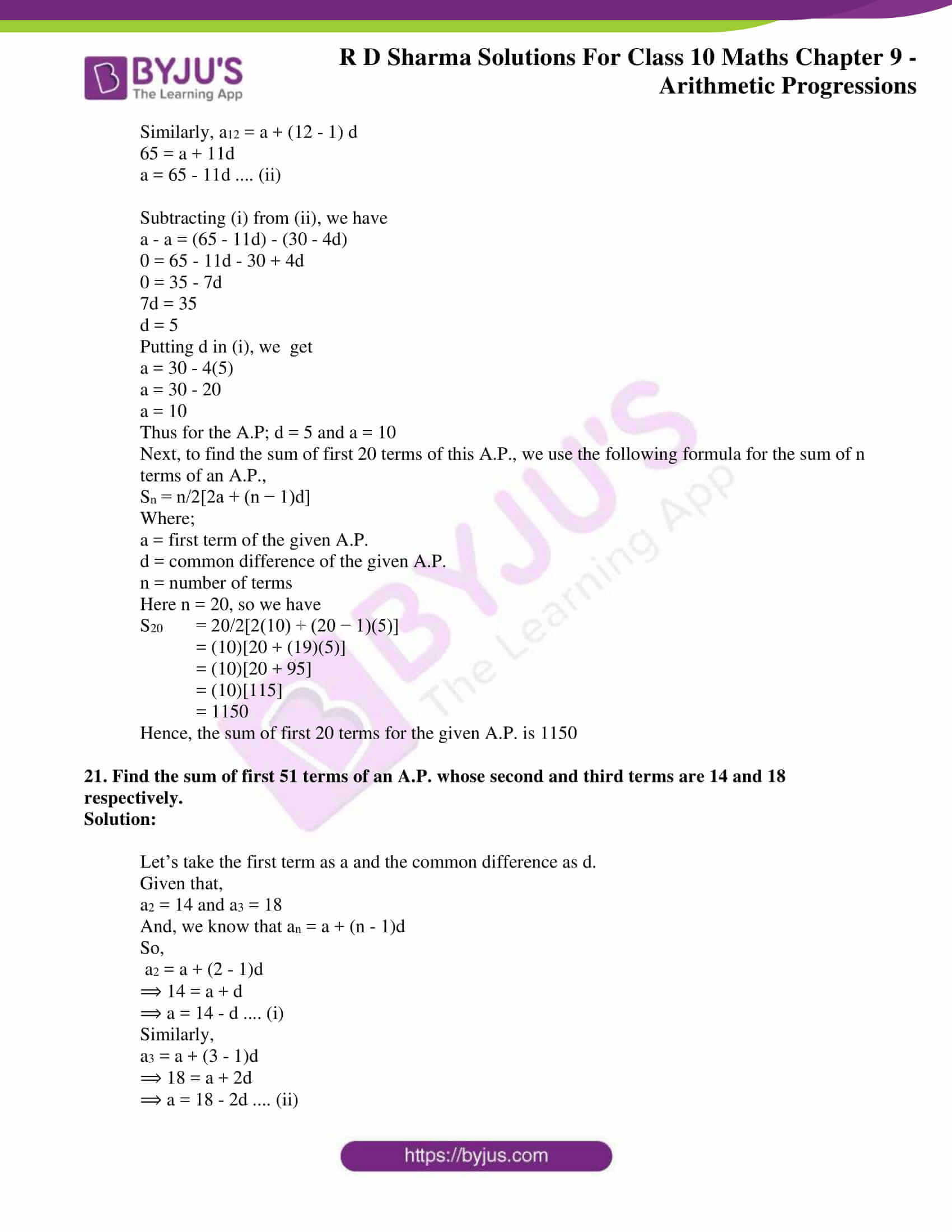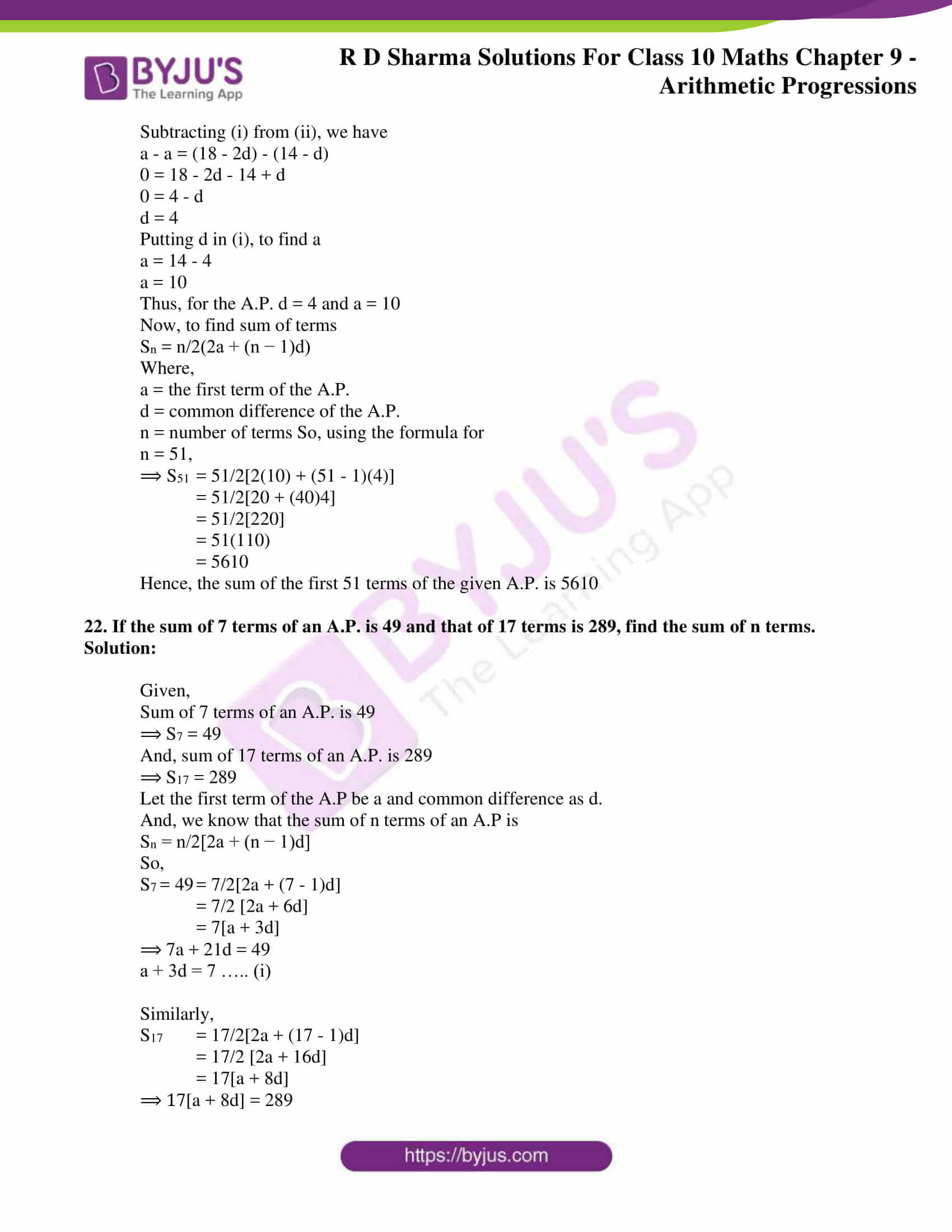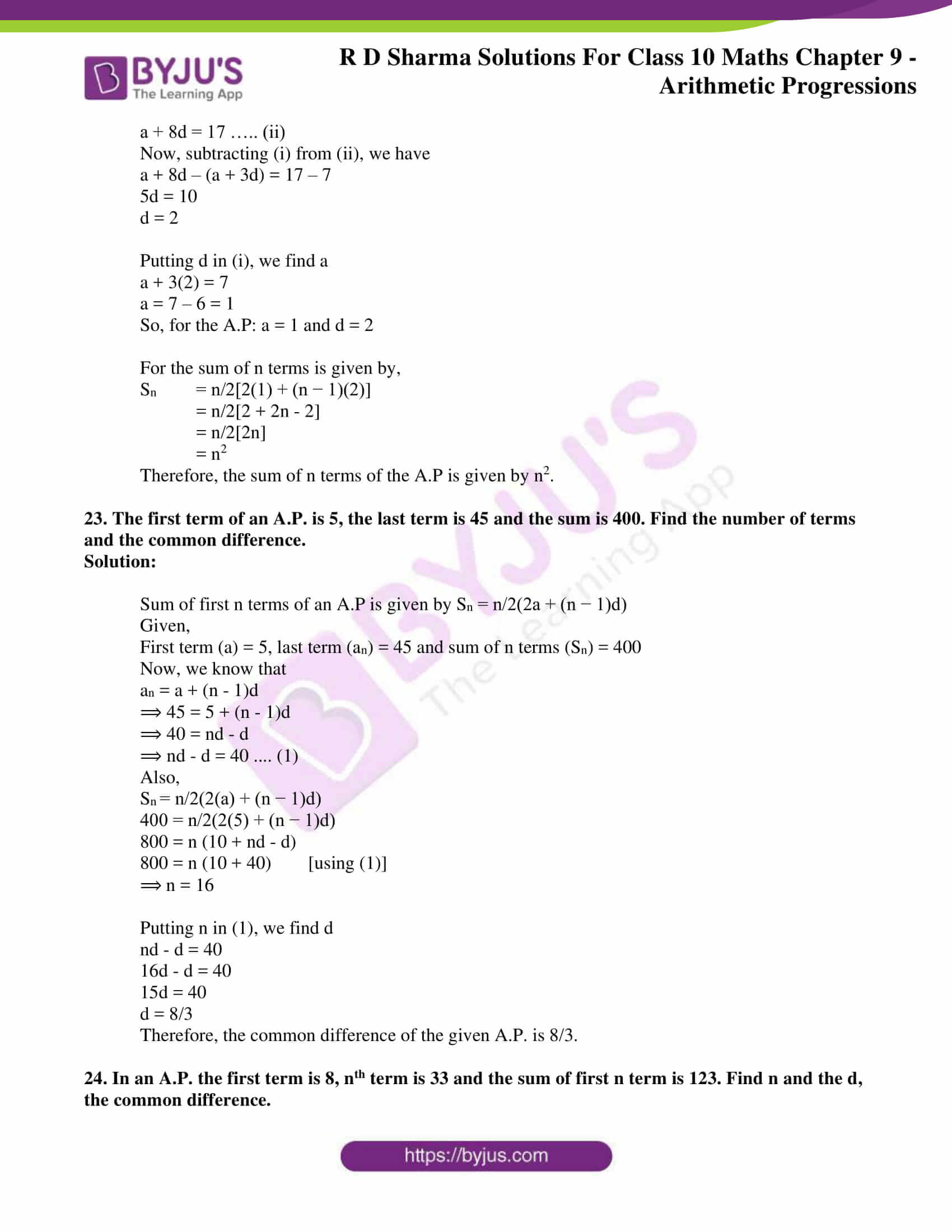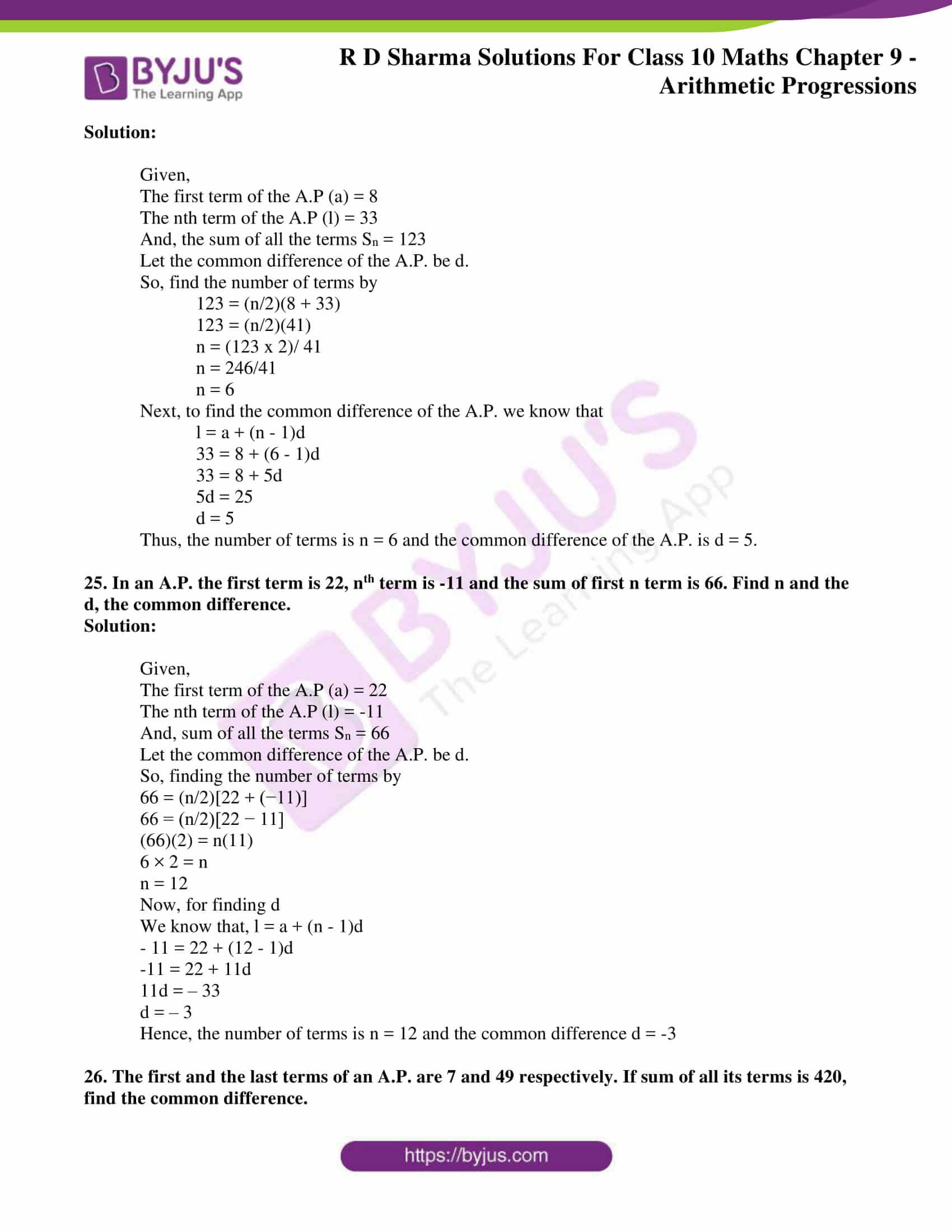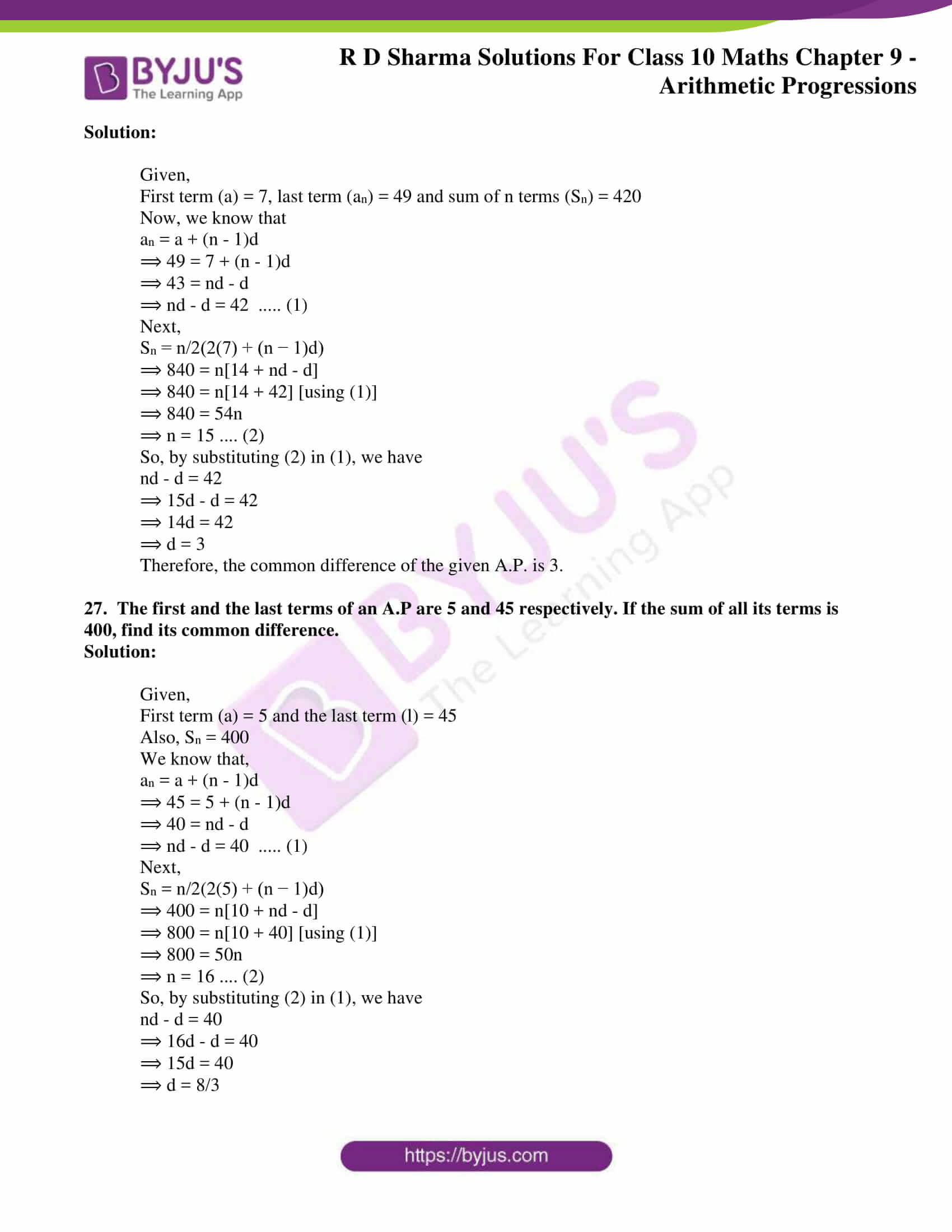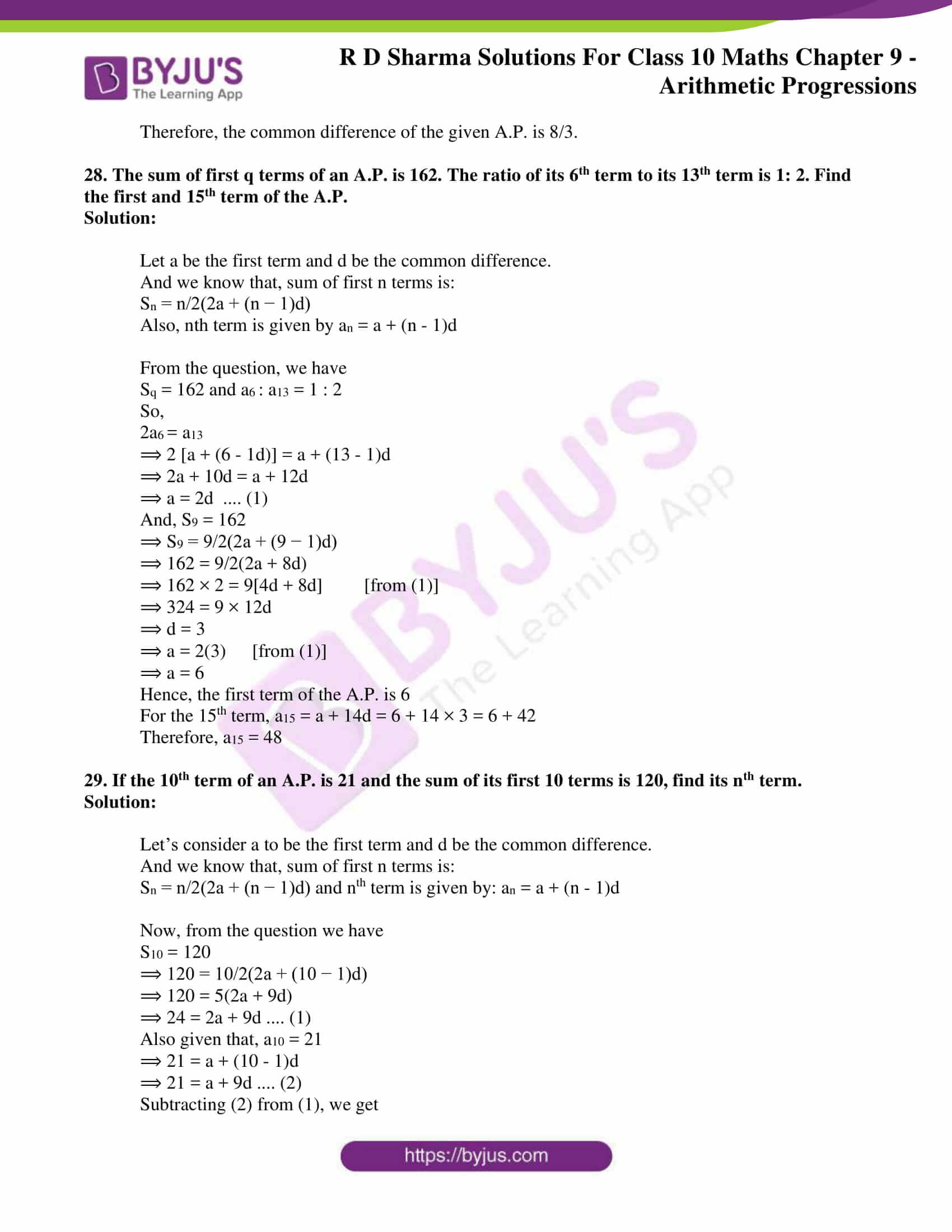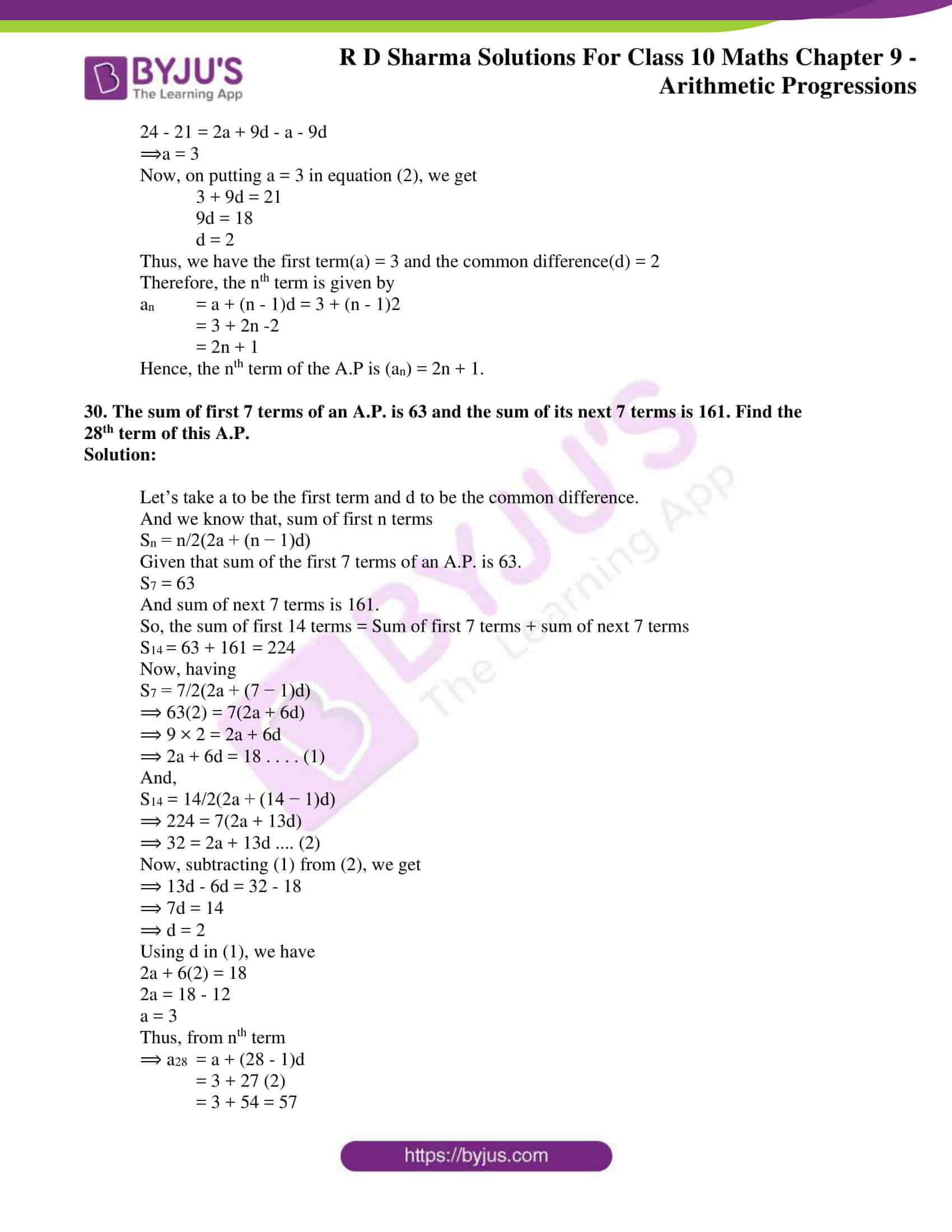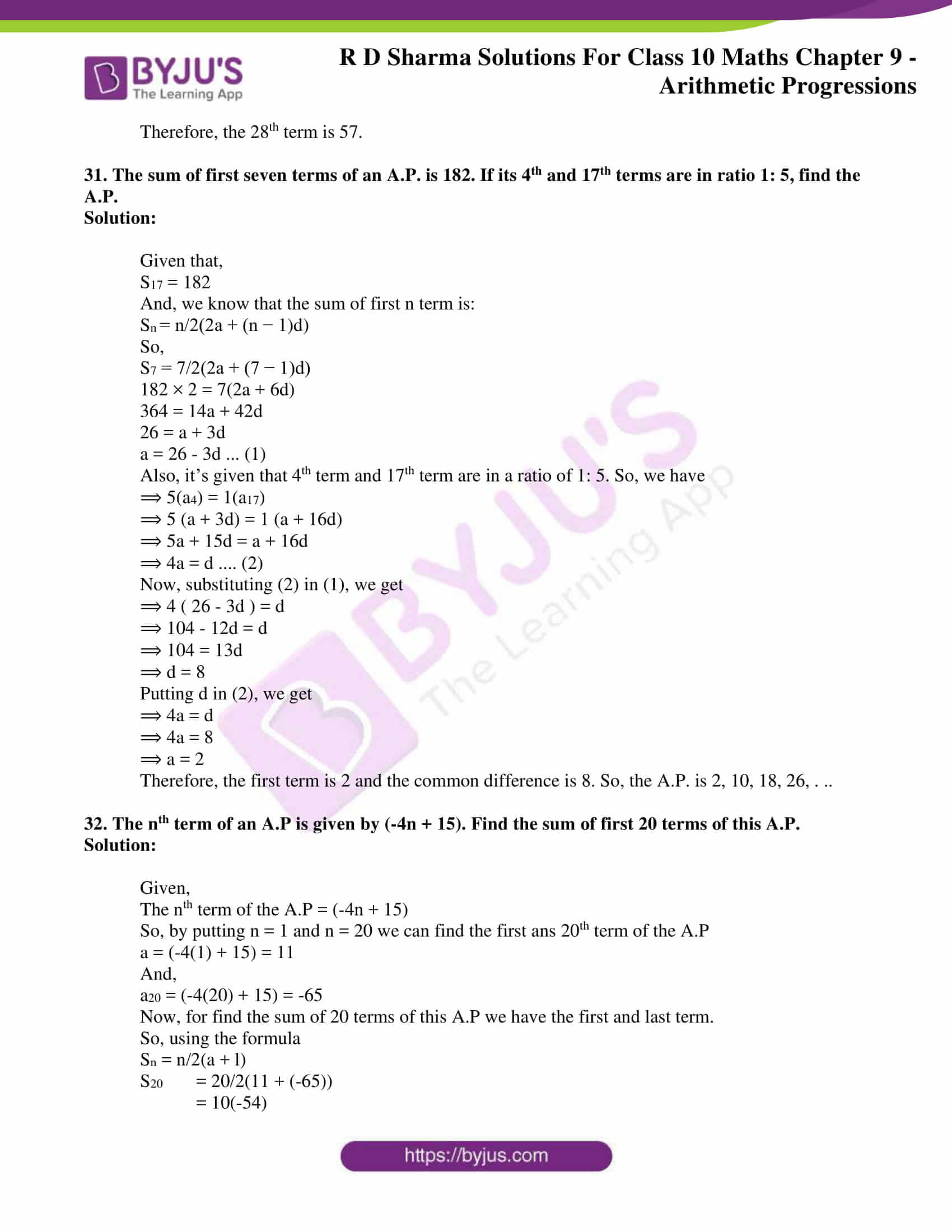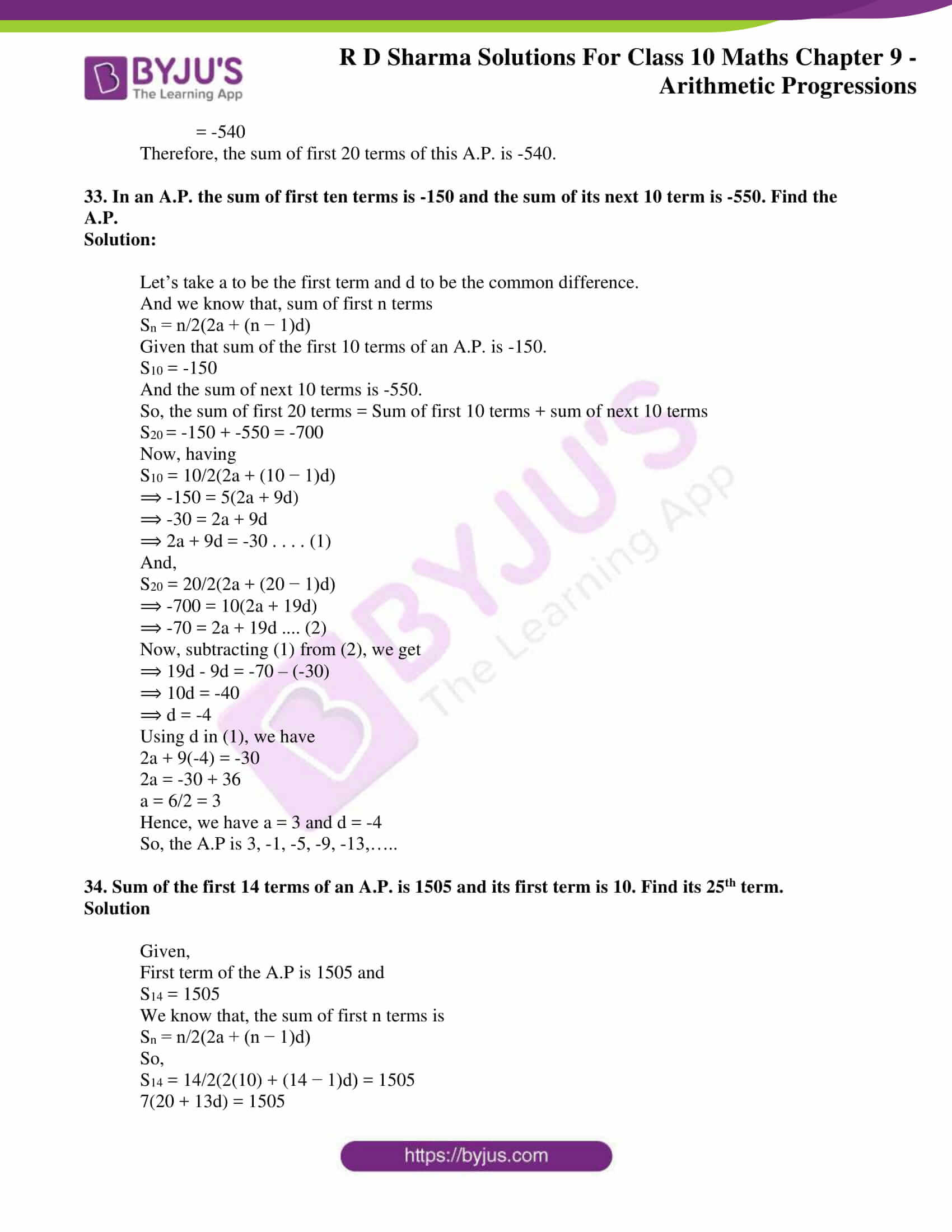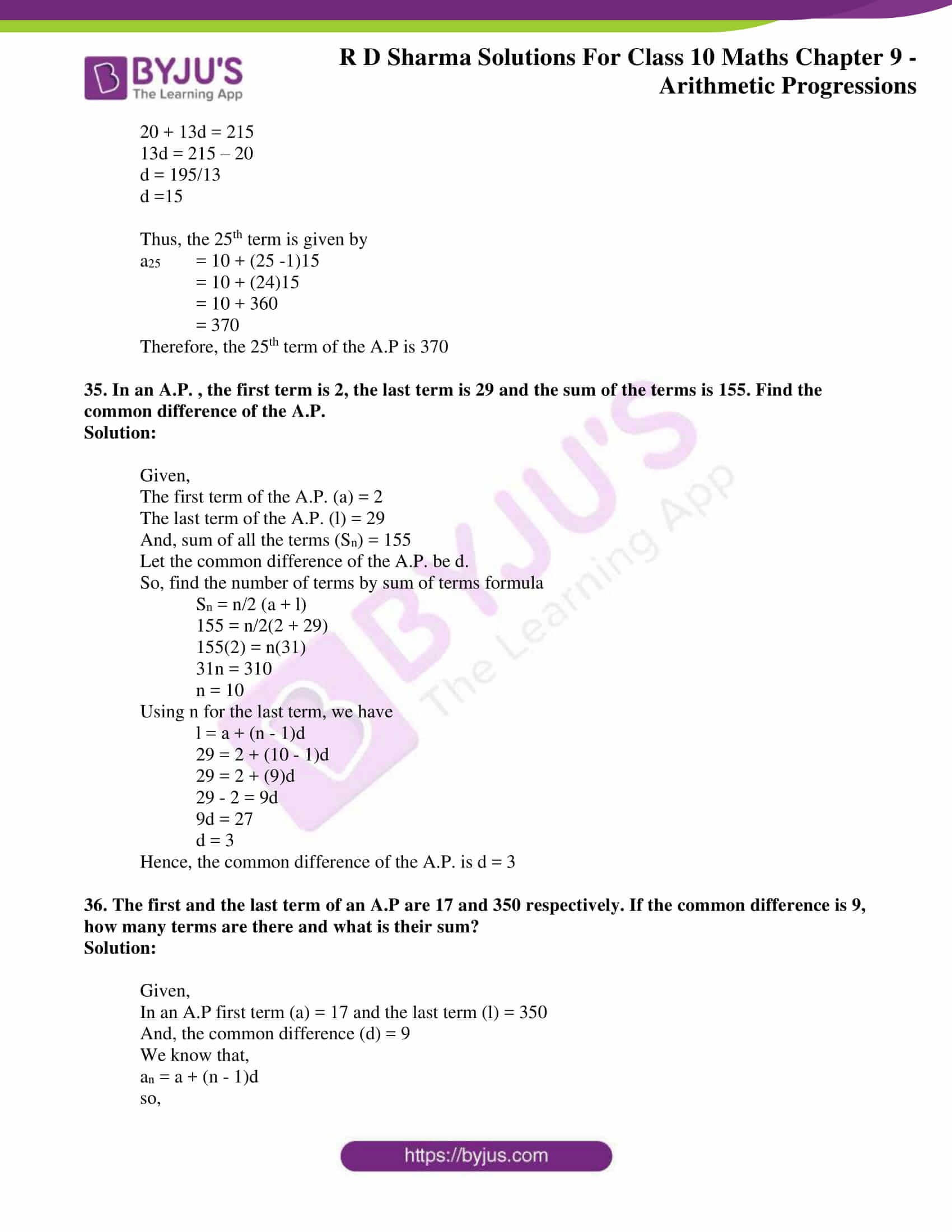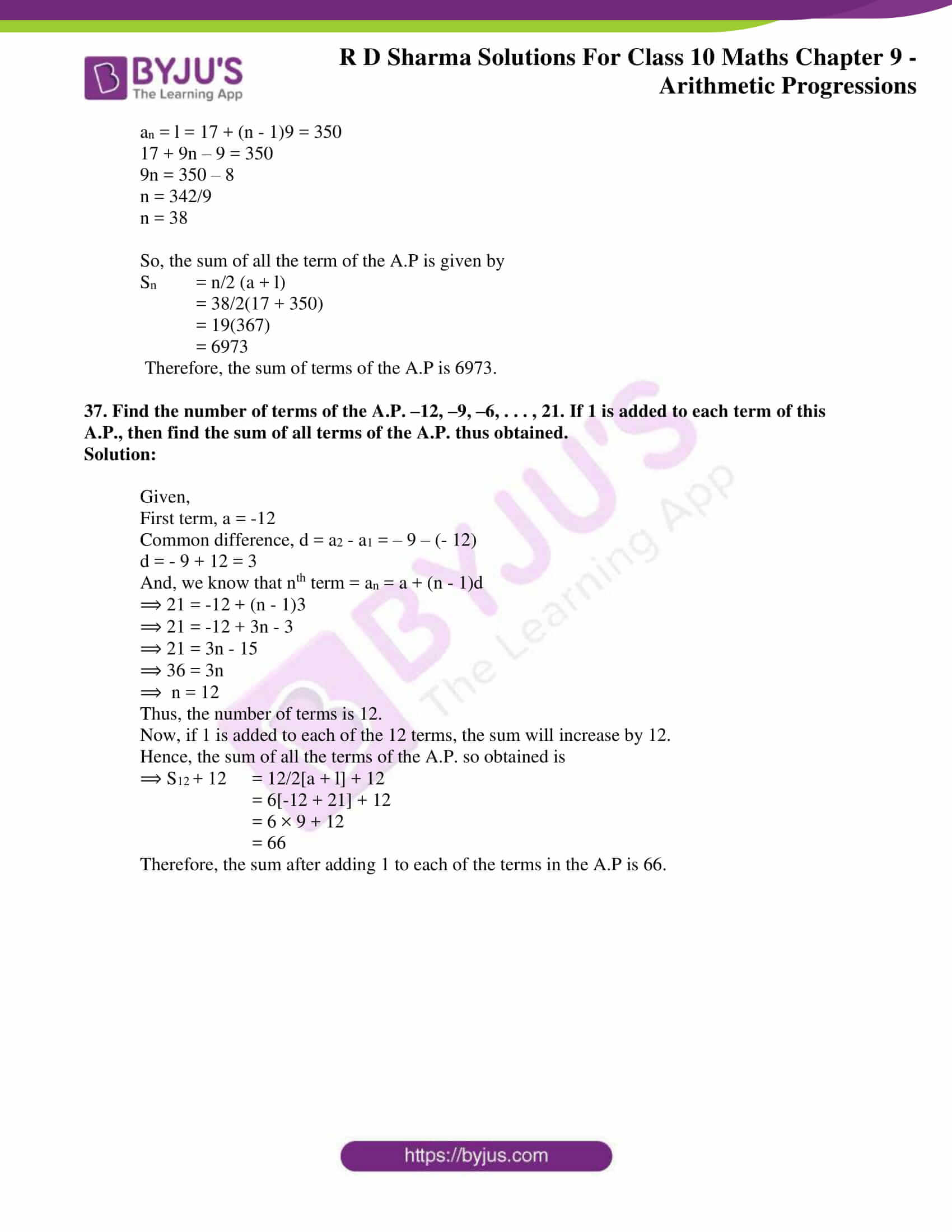### Access RD Sharma Solutions for Class 10 Maths Chapter 9 Arithmetic Progressions Exercise 9.6

1. Find the sum of the following arithmetic progressions:

(i) 50, 46, 42, … to 10 terms

(ii) 1, 3, 5, 7, … to 12 terms

(iii) 3, 9/2, 6, 15/2, … to 25 terms

(iv) 41, 36, 31, … to 12 terms

(v) a + b, a – b, a – 3b, … to 22 terms

(vi) (x – y)2, (x2 + y2), (x + y)2, to 22 tams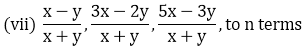(viii) – 26, – 24, – 22, …. to 36 terms

Solution:

In an A.P if the first term = a, common difference = d, and if there are n terms.

Then, sum of n terms is given by: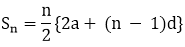(i) Given A.P.is 50, 46, 42 to 10 term.

First term (a) = 50

Common difference (d) = 46 – 50 = – 4

nth term (n) = 10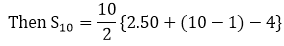= 5{100 – 9.4}

= 5{100 – 36}

= 5 × 64

∴ S10 = 320

(ii) Given A.P is, 1, 3, 5, 7, …..to 12 terms.

First term (a) = 1

Common difference (d) = 3 – 1 = 2

nth term (n) = 12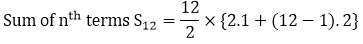= 6 × {2 + 22} = 6.24

∴ S12 = 144

(iii) Given A.P. is 3, 9/2, 6, 15/2, … to 25 terms

First term (a) = 3

Common difference (d) = 9/2 – 3 = 3/2

Sum of n terms Sn, given n = 25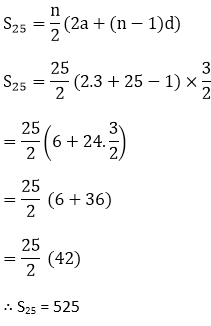(iv) Given expression is 41, 36, 31, ….. to 12 terms.

First term (a) = 41

Common difference (d) = 36 – 41 = -5

Sum of n terms Sn, given n = 12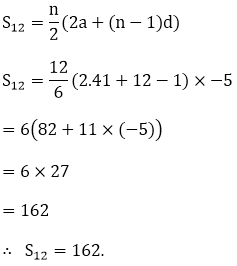(v) a + b, a – b, a – 3b, ….. to 22 terms

First term (a) = a + b

Common difference (d) = a – b – a – b = -2b

Sum of n terms Sn = n/2{2a(n – 1). d}

Here n = 22

S22 = 22/2{2.(a + b) + (22 – 1). -2b}

= 11{2(a + b) – 22b)

= 11{2a – 20b}

= 22a – 440b

∴S22 = 22a – 440b

(vi) (x – y)2,(x2 + y2), (x + y)2,… to n terms

First term (a) = (x – y)2

Common difference (d) = x2 + y2 – (x – y)2

= x2 + y2 – (x2 + y2 – 2xy)

= x2 + y2 – x2 + y2 + 2xy

= 2xy

Sum of nth terms Sn = n/2{2a + (n – 1). d}

= n/2{2(x – y)2 + (n – 1). 2xy}

= n{(x – y)2 + (n – 1)xy}

∴ Sn = n{(x — y)2 + (n — 1). xy)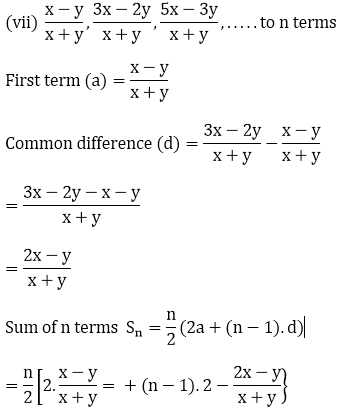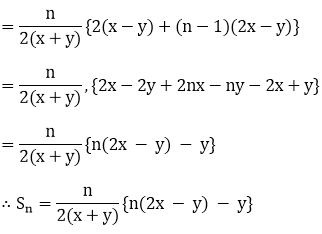(viii) Given expression -26, – 24. -22, to 36 terms

First term (a) = -26

Common difference (d) = -24 – (-26)

= -24 + 26 = 2

Sum of n terms, Sn = n/2{2a + (n – 1)d) for n = 36

Sn = 36/2{2(-26) + (36 – 1)2}

= 18[-52 + 70]

= 18×18

= 324

∴ Sn = 324

2. Find the sum to n terms of the A.P. 5, 2, –1, – 4, –7, …

Solution:

Given AP is 5, 2, -1, -4, -7, …..

Here, a = 5, d = 2 – 5 = -3

We know that,

Sn = n/2{2a + (n – 1)d}

= n/2{2.5 + (n – 1) – 3}

= n/2{10 – 3(n – 1)}

= n/2{13 – 3n)

∴ Sn = n/2(13 – 3n)

3. Find the sum of n terms of an A.P. whose the terms is given by an = 5 – 6n.

Solution:

Given nth term of the A.P as an = 5 – 6n

Put n = 1, we get

a1 = 5 – 6.1 = -1

So, first term (a) = -1

Last term (an) = 5 – 6n = 1

Then, Sn = n/2(-1 + 5 – 6n)

= n/2(4 – 6n) = n(2 – 3n)

4. Find the sum of last ten terms of the A.P. : 8, 10, 12, 14, .. , 126

Solution:

Given A.P. 8, 10, 12, 14, .. , 126

Here, a = 8 , d = 10 – 8 = 2

We know that, an = a + (n – 1)d

So, to find the number of terms

126 = 8 + (n – 1)2

126 = 8 + 2n – 2

2n = 120

n = 60

Next, let’s find the 51st term

a51 = 8 + 50(2) = 108

So, the sum of last ten terms is the sum of a51 + a52 + a53 + ……. + a60

Here, n = 10, a = 108 and l = 126

S = 10/2 [108 + 126]

= 5(234)

= 1170

Hence, the sum of last ten terms of the A.P is 1170.

5. Find the sum of first 15 terms of each of the following sequences having nth term as:

(i) an = 3 + 4n

(ii) bn = 5 + 2n

(iii) xn = 6 – n

(iv) yn = 9 – 5n

Solution:

(i) Given an A.P. whose nth term is given by an = 3 + 4n

To find the sum of the n terms of the given A.P., using the formula,

Sn = n(a + l)/ 2

Where, a = the first term l = the last term.

Putting n = 1 in the given an, we get

a = 3 + 4(1) = 3 + 4 = 7

For the last term (l), here n = 15

a15 = 3 + 4(15) = 63

So, Sn = 15(7 + 63)/2

= 15 x 35

= 525

Therefore, the sum of the 15 terms of the given A.P. is S15 = 525

(ii) Given an A.P. whose nth term is given by bn = 5 + 2n

To find the sum of the n terms of the given A.P., using the formula,

Sn = n(a + l)/ 2

Where, a = the first term l = the last term.

Putting n = 1 in the given bn, we get

a = 5 + 2(1) = 5 + 2 = 7

For the last term (l), here n = 15

a15 = 5 + 2(15) = 35

So, Sn = 15(7 + 35)/2

= 15 x 21

= 315

Therefore, the sum of the 15 terms of the given A.P. is S15 = 315

(iii) Given an A.P. whose nth term is given by xn = 6 – n

To find the sum of the n terms of the given A.P., using the formula

Sn = n(a + l)/ 2

Where, a = the first term l = the last term.

Putting n = 1 in the given xn, we get

a = 6 – 1 = 5

For the last term (l), here n = 15

a15 = 6 – 15 = -9

So, Sn = 15(5 – 9)/2

= 15 x (-2)

= -30

Therefore, the sum of the 15 terms of the given A.P. is S15 = -30

(iv) Given an A.P. whose nth term is given by yn = 9 – 5n

To find the sum of the n terms of the given A.P., using the formula,

Sn = n(a + l)/ 2

Where, a = the first term l = the last term.

Putting n = 1 in the given yn, we get

a = 9 – 5(1) = 9 – 5 = 4

For the last term (l), here n = 15

a15 = 9 – 5(15) = -66

So, Sn = 15(4 – 66)/2

= 15 x (-31)

= -465

Therefore, the sum of the 15 terms of the given A.P. is S15 = -465

6. Find the sum of first 20 terms the sequence whose nth term is an = An + B.

Solution:

Given an A.P. whose nth term is given by, an = An + B

We need to find the sum of first 20 terms.

To find the sum of the n terms of the given A.P., we use the formula,

Sn = n(a + l)/ 2

Where, a = the first term l = the last term,

Putting n = 1 in the given an, we get

a = A(1) + B = A + B

For the last term (l), here n = 20

A20 = A(20) + B = 20A + B

S20 = 20/2((A + B) + 20A + B)

= 10[21A + 2B]

= 210A + 20B

Therefore, the sum of the first 20 terms of the given A.P. is 210 A + 20B

7. Find the sum of first 25 terms of an A.P whose nth term is given by an = 2 – 3n.

Solution:

Given an A.P. whose nth term is given by an = 2 – 3n

To find the sum of the n terms of the given A.P., we use the formula,

Sn = n(a + l)/ 2

Where, a = the first term l = the last term.

Putting n = 1 in the given an, we get

a = 2 – 3(1) = -1

For the last term (l), here n = 25

a25 = 2 – 3(25) = -73

So, Sn = 25(-1 – 73)/2

= 25 x (-37)

= -925

Therefore, the sum of the 25 terms of the given A.P. is S25 = -925

8. Find the sum of first 25 terms of an A.P whose nth term is given by an = 7 – 3n.

Solution:

Given an A.P. whose nth term is given by an = 7 – 3n

To find the sum of the n terms of the given A.P., we use the formula,

Sn = n(a + l)/ 2

Where, a = the first term l = the last term.

Putting n = 1 in the given an, we get

a = 7 – 3(1) = 7 – 3 = 4

For the last term (l), here n = 25

a15 = 7 – 3(25) = -68

So, Sn = 25(4 – 68)/2

= 25 x (-32)

= -800

Therefore, the sum of the 15 terms of the given A.P. is S25 = -800

9. If the sum of a certain number of terms starting from first term of an A.P. is 25, 22, 19, . . ., is 116. Find the last term.

Solution:

Given the sum of the certain number of terms of an A.P. = 116

We know that, Sn = n/2[2a + (n − 1)d]

Where; a = first term for the given A.P.

d = common difference of the given A.P.

n = number of terms So for the given A.P.(25, 22, 19,…)

Here we have, the first term (a) = 25

The sum of n terms Sn = 116

Common difference of the A.P. (d) = a2 – a1 = 22 – 25 = -3

Now, substituting values in Sn

⟹ 116 = n/2[2(25) + (n − 1)(−3)]

⟹ (n/2)[50 + (−3n + 3)]  = 116

⟹ (n/2)[53 − 3n] = 116

⟹ 53n – 3n2 = 116 x 2

Thus, we get the following quadratic equation,

3n2 – 53n + 232 = 0

By factorization method of solving, we have

⟹ 3n2 – 24n – 29n + 232 = 0

⟹ 3n( n – 8 ) – 29 ( n – 8 ) = 0

⟹ (3n – 29)( n – 8 ) = 0

So, 3n – 29 = 0

⟹ n = 29/3

Also, n – 8 = 0

⟹ n = 8

Since, n cannot be a fraction, so the number of terms is taken as 8.

So, the term is:

a8 = a1 + 7d = 25 + 7(-3) = 25 – 21 = 4

Hence, the last term of the given A.P. such that the sum of the terms is 116 is 4.

10. (i) How many terms of the sequence 18, 16, 14….  should be taken so that their sum is zero.

(ii) How many terms are there in the A.P. whose first and fifth terms are -14 and 2 respectively and the sum of the terms is 40?

(iii) How many terms of the A.P. 9, 17, 25, . . . must be taken so that their sum is 636?

(iv) How many terms of the A.P. 63, 60, 57, . . . must be taken so that their sum is 693?

(v) How many terms of the A.P. is 27, 24, 21. . . should be taken that their sum is zero?

Solution:

(i) Given AP. is 18, 16, 14, …

We know that,

Sn = n/2[2a + (n − 1)d]

Here,

The first term (a) = 18

The sum of n terms (Sn) = 0 (given)

Common difference of the A.P.

(d) = a2  – a1 = 16 – 18 = – 2

So, on substituting the values in Sn

⟹ 0 = n/2[2(18) + (n − 1)(−2)]

⟹ 0 = n/2[36 + (−2n + 2)]

⟹ 0 = n/2[38 − 2n] Further, n/2

⟹ n = 0 Or, 38 – 2n = 0

⟹ 2n = 38

⟹ n = 19

Since, the number of terms cannot be zer0, hence the number of terms (n) should be 19.

(ii) Given, the first term (a) = -14, Filth term (a5) = 2, Sum of terms (Sn) = 40 of the A.P.

If the common difference is taken as d.

Then, a5 = a + 4d

⟹ 2 = -14 + 4d

⟹ 2 + 14 = 4d

⟹ 4d = 16

⟹ d = 4

Next, we know that S= n/2[2a + (n − 1)d]

Where; a = first term for the given A.P.

d = common difference of the given A.P.

n = number of terms

Now, on substituting the values in Sn

⟹ 40 = n/2[2(−14) + (n − 1)(4)]

⟹ 40 = n/2[−28 + (4n − 4)]

⟹ 40 = n/2[−32 + 4n]

⟹ 40(2) = – 32n + 4n2

So, we get the following quadratic equation,

4n2 – 32n – 80 = 0

⟹ n2 – 8n – 20 = 0

On solving by factorization method, we get

n2 – 10n + 2n – 20 = 0

⟹ n(n – 10) + 2(n – 10 ) = 0

⟹ (n + 2)(n – 10) = 0

Either, n + 2 = 0

⟹ n = -2

Or, n – 10 = 0

⟹ n = 10

Since the number of terms cannot be negative.

Therefore, the number of terms (n) is 10.

(iii) Given AP is 9, 17, 25,…

We know that,

Sn = n/2[2a + (n − 1)d]

Here we have,

The first term (a) = 9 and the sum of n terms (Sn) = 636

Common difference of the A.P. (d) = a2  – a1 = 17 – 9 = 8

Substituting the values in Sn, we get

⟹ 636 = n/2[2(9) + (n − 1)(8)]

⟹ 636 = n/2[18 + (8n − 8)]

⟹ 636(2) = (n)[10 + 8n]

⟹ 1271 = 10n + 8n2

Now, we get the following quadratic equation,

⟹ 8n2 + 10n – 1272 = 0

⟹ 4n2+ 5n – 636 = 0

On solving by factorisation method, we have

⟹ 4n2 – 48n + 53n – 636 = 0

⟹ 4n(n – 12) + 53(n – 12) = 0

⟹ (4n + 53)(n – 12) = 0

Either 4n + 53 = 0 ⟹ n = -53/4

Or, n – 12 = 0 ⟹ n = 12

Since, the number of terms cannot be a fraction.

Therefore, the number of terms (n) is 12.

(iv) Given A.P. is 63, 60, 57,…

We know that,

Sn = n/2[2a + (n − 1)d]

Here we have,

the first term (a) = 63

The sum of n terms (Sn) = 693

Common difference of the A.P. (d) = a2 – a1 = 60 – 63 = –3

On substituting the values in Sn we get

⟹ 693 = n/2[2(63) + (n − 1)(−3)]

⟹ 693 = n/2[126+(−3n + 3)]

⟹ 693 = n/2[129 − 3n]

⟹ 693(2) = 129n – 3n2

Now, we get the following quadratic equation.

⟹ 3n2 – 129n + 1386 = 0

⟹ n2 – 43n + 462

Solving by factorisation method, we have

⟹ n2 – 22n – 21n + 462 = 0

⟹ n(n – 22) -21(n – 22) = 0

⟹ (n – 22) (n – 21) = 0

Either, n – 22 = 0 ⟹ n = 22

Or,  n – 21 = 0 ⟹ n = 21

Now, the 22nd term will be a22 = a1 + 21d = 63 + 21( -3 ) = 63 – 63 = 0

So, the sum of 22 as well as 21 terms is 693.

Therefore, the number of terms (n) is 21 or 22.

(v) Given A.P. is 27, 24, 21. . .

We know that,

Sn = n/2[2a + (n − 1)d]

Here we have, the first term (a) = 27

The sum of n terms (Sn) = 0

Common difference of the A.P. (d) = a2 – a1 = 24 – 27 = -3

On substituting the values in Sn, we get

⟹ 0 = n/2[2(27) + (n − 1)( − 3)]

⟹ 0 = (n)[54 + (n – 1)(-3)]

⟹ 0 = (n)[54 – 3n + 3]

⟹ 0 = n [57 – 3n] Further we have, n = 0 Or, 57 – 3n = 0

⟹ 3n = 57

⟹ n = 19

The number of terms cannot be zero,

Hence, the numbers of terms (n) is 19.

11. Find the sum of the first

(i) 11 terms of the A.P. : 2, 6, 10, 14,  . . .

(ii) 13 terms of the A.P. : -6, 0, 6, 12, . . .

(iii) 51 terms of the A.P. : whose second term is 2 and fourth term is 8.

Solution:

We know that the sum of terms for different arithmetic progressions is given by

Sn = n/2[2a + (n − 1)d]

Where; a = first term for the given A.P. d = common difference of the given A.P. n = number of terms

(i) Given A.P 2, 6, 10, 14,… to 11 terms.

Common difference (d) = a2 – a1 = 10 – 6 = 4

Number of terms (n) = 11

First term for the given A.P. (a) = 2

So,

S11  = 11/2[2(2) + (11 − 1)4]

= 11/2[2(2) + (10)4]

= 11/2[4 + 40]

= 11 × 22

= 242

Hence, the sum of first 11 terms for the given A.P. is 242

(ii) Given A.P. – 6, 0, 6, 12, … to 13 terms.

Common difference (d) = a2 – a1 = 6 – 0 = 6

Number of terms (n) = 13

First term (a) = -6

So,

S13  = 13/2[2(− 6) + (13 –1)6]

= 13/2[(−12) + (12)6]

= 13/2 = 390

Hence, the sum of first 13 terms for the given A.P. is 390

(iii) 51 terms of an AP whose a2 = 2 and a4 = 8

We know that, a2 = a + d

2 = a + d  …(2)

Also, a4 = a + 3d

8 = a + 3d  … (2)

Subtracting (1) from (2), we have

2d = 6

d = 3

Substituting d = 3 in (1), we get

2 = a + 3

⟹ a = -1

Given that the number of terms (n) = 51

First term (a) = -1

So,

Sn  = 51/2[2(−1) + (51 − 1)(3)]

= 51/2[−2 + 150]

= 51/2

= 3774

Hence, the sum of first 51 terms for the A.P. is 3774.

12. Find the sum of

(i) the first 15 multiples of 8

(ii) the first 40 positive integers divisible by (a) 3 (b) 5 (c) 6.

(iii) all 3 – digit natural numbers which are divisible by 13.

(iv) all 3 – digit natural numbers which are multiples of 11.

Solution:

We know that the sum of terms for an A.P is given by

Sn = n/2[2a + (n − 1)d]

Where; a = first term for the given A.P. d = common difference of the given A.P. n = number of terms

(i) Given, first 15 multiples of 8.

These multiples form an A.P: 8, 16, 24, …… , 120

Here, a = 8 , d = 61 – 8 = 8 and the number of terms(n) = 15

Now, finding the sum of 15 terms, we have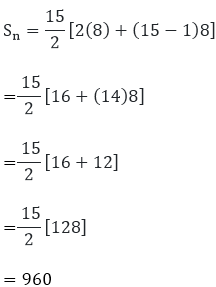\

Hence, the sum of the first 15 multiples of 8 is 960

(ii)(a) First 40 positive integers divisible by 3.

Hence, the first multiple is 3 and the 40th multiple is 120.

And, these terms will form an A.P. with the common difference of 3.

Here, First term (a) = 3

Number of terms (n) = 40

Common difference (d) = 3

So, the sum of 40 terms

S40  = 40/2[2(3) + (40 − 1)3]

= 20[6 + (39)3]

= 20(6 + 117)

= 20(123) = 2460

Thus, the sum of first 40 multiples of 3 is 2460.

(b) First 40 positive integers divisible by 5

Hence, the first multiple is 5 and the 40th multiple is 200.

And, these terms will form an A.P. with the common difference of 5.

Here, First term (a) = 5

Number of terms (n) = 40

Common difference (d) = 5

So, the sum of 40 terms

S40  = 40/2[2(5) + (40 − 1)5]

= 20[10 + (39)5]

= 20 (10 + 195)

= 20 (205) = 4100

Hence, the sum of first 40 multiples of 5 is 4100.

(c) First 40 positive integers divisible by 6

Hence, the first multiple is 6 and the 40th multiple is 240.

And, these terms will form an A.P. with the common difference of 6.

Here, First term (a) = 6

Number of terms (n) = 40

Common difference (d) = 6

So, the sum of 40 terms

S40  = 40/2[2(6) + (40 − 1)6]

= 20[12 + (39)6]

=20(12 + 234)

= 20(246) = 4920

Hence, the sum of first 40 multiples of 6 is 4920.

(iii) All 3 digit natural number which are divisible by 13.

So, we know that the first 3 digit multiple of 13 is 104 and the last 3 digit multiple of 13 is 988.

And, these terms form an A.P. with the common difference of 13.

Here, first term (a) = 104 and the last term (l) = 988

Common difference (d) = 13

Finding the number of terms in the A.P. by, an = a + (n − 1)d

We have,

988 = 104 + (n – 1)13

⟹ 988 = 104 + 13n -13

⟹ 988 = 91 + 13n

⟹ 13n = 897

⟹ n = 69

Now, using the formula for the sum of n terms, we get

S69  = 69/2[2(104) + (69 − 1)13]

= 69/2[208 + 884]

= 69/2

= 69(546)

= 37674

Hence, the sum of all 3 digit multiples of 13 is 37674.

(iv) All 3 digit natural number which are multiples of 11.

So, we know that the first 3 digit multiple of 11 is 110 and the last 3 digit multiple of 13 is 990.

And, these terms form an A.P. with the common difference of 11.

Here, first term (a) = 110 and the last term (l) = 990

Common difference (d) = 11

Finding the number of terms in the A.P. by, an = a + (n − 1)d

We get,

990 = 110 + (n – 1)11

⟹ 990 = 110 + 11n -11

⟹ 990 = 99 + 11n

⟹ 11n = 891

⟹ n = 81

Now, using the formula for the sum of n terms, we get

S81  = 81/2[2(110) + (81 − 1)11]

= 81/2[220 + 880]

= 81/2

= 81(550)

= 44550

Hence, the sum of all 3 digit multiples of 11 is 44550.

13. Find the sum:

(i) 2 + 4 + 6 + . . . + 200

(ii) 3 + 11 + 19 + . . . + 803

(iii) (-5) + (-8) + (-11) + . . . + (- 230)

(iv) 1 + 3 + 5 + 7 + . . . + 199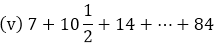(vi) 34 + 32 + 30 + . . . + 10

(vii) 25 + 28 + 31 + . . . + 100

Solution:

We know that the sum of terms for an A.P is given by

Sn = n/2[2a + (n − 1)d]

Where; a = first term for the given A.P. d = common difference of the given A.P. n = number of terms

Or Sn = n/2[a + l]

Where; a = first term for the given A.P. ;l = last term for the given A.P

(i) Given series. 2 + 4 + 6 + . . . + 200 which is an A.P

Where, a = 2 ,d = 4 – 2 = 2 and last term (an = l) = 200

We know that, an = a + (n – 1)d

So,

200 = 2 + (n – 1)2

200 = 2 + 2n – 2

n = 200/2 = 100

Now, for the sum of these 100 terms

S100 = 100/2 [2 + 200]

= 50(202)

= 10100

Hence, the sum of terms of the given series is 10100.

(ii) Given series. 3 + 11 + 19 + . . . + 803 which is an A.P

Where, a = 3 ,d = 11 – 3 = 8 and last term (an = l) = 803

We know that, an = a + (n – 1)d

So,

803 = 3 + (n – 1)8

803 = 3 + 8n – 8

n = 808/8 = 101

Now, for the sum of these 101 terms

S101 = 101/2 [3 + 803]

= 101(806)/2

= 101 x 403

= 40703

Hence, the sum of terms of the given series is 40703.

(iii) Given series (-5) + (-8) + (-11) + . . . + (- 230) which is an A.P

Where, a = -5 ,d = -8 – (-5) = -3 and last term (an = l) = -230

We know that, an = a + (n – 1)d

So,

-230 = -5 + (n – 1)(-3)

-230 = -5 – 3n + 3

3n = -2 + 230

n = 228/3 = 76

Now, for the sum of these 76 terms

S76 = 76/2 [-5 + (-230)]

= 38 x (-235)

= -8930

Hence, the sum of terms of the given series is -8930.

(iv) Given series. 1 + 3 + 5 + 7 + . . . + 199 which is an A.P

Where, a = 1 ,d = 3 – 1 = 2 and last term (an = l) = 199

We know that, an = a + (n – 1)d

So,

199 = 1 + (n – 1)2

199 = 1 + 2n – 2

n = 200/2 = 100

Now, for the sum of these 100 terms

S100 = 100/2 [1 + 199]

= 50(200)

= 10000

Hence, the sum of terms of the given series is 10000.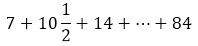(v) Given series which is an A.P

Where, a = 7, d = 10 ½ – 7 = (21 – 14)/2 = 7/2 and last term (an = l) = 84

We know that, an = a + (n – 1)d

So,

84 = 7 + (n – 1)(7/2)

168 = 14 + 7n – 7

n = (168 – 7)/7 = 161/7 = 23

Now, for the sum of these 23 terms

S23 = 23/2 [7 + 84]

= 23(91)/2

= 2093/2

Hence, the sum of terms of the given series is 2093/2.

(vi) Given series, 34 + 32 + 30 + . . . + 10 which is an A.P

Where, a = 34 ,d = 32 – 34 = -2 and last term (an = l) = 10

We know that, an = a + (n – 1)d

So,

10 = 34 + (n – 1)(-2)

10 = 34 – 2n + 2

n = (36 – 10)/2 = 13

Now, for the sum of these 13 terms

S13 = 13/2 [34 + 10]

= 13(44)/2

= 13 x 22

= 286

Hence, the sum of terms of the given series is 286.

(vii) Given series, 25 + 28 + 31 + . . . + 100 which is an A.P

Where, a = 25 ,d = 28 – 25 = 3 and last term (an = l) = 100

We know that, an = a + (n – 1)d

So,

100 = 25 + (n – 1)(3)

100 = 25 + 3n – 3

n = (100 – 22)/3 = 26

Now, for the sum of these 26 terms

S100 = 26/2 [25 + 100]

= 13(125)

= 1625

Hence, the sum of terms of the given series is 1625.

14. The first and the last terms of an A.P. are 17 and 350 respectively. If the common difference is 9, how many terms are there and what is their sum?

Solution:

Given, the first term of the A.P (a) = 17

The last term of the A.P (l) = 350

The common difference (d) of the A.P. = 9

Let the number of terms be n. And, we know that; l = a + (n – 1)d

So, 350 = 17 + (n- 1) 9

⟹ 350 = 17 + 9n – 9

⟹ 350 = 8 + 9n

⟹ 350 – 8 = 9n

Thus we get, n = 38

Now, finding the sum of terms

Sn  = n/2[a + l]

= 38/2(17 + 350)

= 19 × 367

= 6973

Hence, the number of terms is of the A.P is 38 and their sum is 6973.

15. The third term of an A.P. is 7 and the seventh term exceeds three times the third term by 2. Find the first term, the common difference and the sum of first 20 terms.

Solution:

Let’s consider the first term as a and the common difference as d.

Given,

a3 = 7 …. (1) and,

a7 = 3a3 + 2   …. (2)

So, using (1) in (2), we get,

a= 3(7) + 2 = 21 + 2 = 23  …. (3)

Also, we know that

an = a +(n – 1)d

So, the 3th term (for n = 3),

a3 = a + (3 – 1)d

⟹ 7 = a + 2d   (Using 1)

⟹ a = 7 – 2d     …. (4)

Similarly, for the 7th term (n = 7),

a7 = a + (7 – 1) d 24 = a + 6d = 23  (Using 3)

a = 23 – 6d  …. (5)

Subtracting (4) from (5), we get,

a – a = (23 – 6d) – (7 – 2d)

⟹ 0 = 23 – 6d – 7 + 2d

⟹ 0 = 16 – 4d

⟹ 4d = 16

⟹ d = 4

Now, to find a, we substitute the value of d in  (4), a =7 – 2(4)

⟹ a = 7 – 8

a = -1

Hence, for the A.P. a = -1 and d = 4

For finding the sum, we know that

Sn = n/2[2a + (n − 1)d] and n = 20 (given)

S20  = 20/2[2(−1) + (20 − 1)(4)]

= (10)[-2 + (19)(4)]

= (10)[-2 + 76]

= (10)

= 740

Hence, the sum of first 20 terms for the given A.P. is 740

16. The first term of an A.P. is 2 and the last term is 50. The sum of all these terms is 442. Find the common difference.

Solution:

Given,

The first term of the A.P (a) = 2

The last term of the A.P (l) = 50

Sum of all the terms Sn = 442

So, let the common difference of the A.P. be taken as d.

The sum of all the terms is given as,

442 = (n/2)(2 + 50)

⟹ 442 = (n/2)(52)

⟹ 26n = 442

⟹ n = 17

Now, the last term is expressed as

50 = 2 + (17 – 1)d

⟹ 50 = 2 + 16d

⟹ 16d = 48

⟹ d = 3

Thus, the common difference of the A.P. is d = 3.

17. If 12th term of an A.P. is -13 and the sum of the first four terms is 24, what is the sum of first 10 terms?

Solution:

Let us take the first term as a and the common difference as d.

Given,

a12 = -13 S4 = 24

Also, we know that a= a + (n – 1)d

So, for the 12th term

a12 = a + (12 – 1)d = -13

⟹ a + 11d = -13

a = -13 – 11d  …. (1)

And, we that for sum of terms

Sn = n/2[2a + (n − 1)d]

Here, n = 4

S4  = 4/2[2(a) + (4 − 1)d]

⟹ 24 = (2)[2a + (3)(d)]

⟹ 24 = 4a + 6d

⟹ 4a = 24 – 6d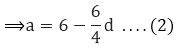Subtracting (1) from (2), we have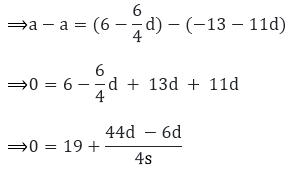Further simplifying for d, we get,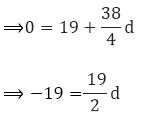⟹ -19 × 2 = 19d

⟹ d = – 2

On substituting the value of d in (1), we find a

a = -13 – 11(-2)

a = -13 + 22

a = 9

Next, the sum of 10 term is given by

S10  = 10/2[2(9) + (10 − 1)(−2)]

= (5)[19 + (9)(-2)]

= (5)(18 – 18) = 0

Thus, the sum of first 10 terms for the given A.P. is S10 = 0.

18.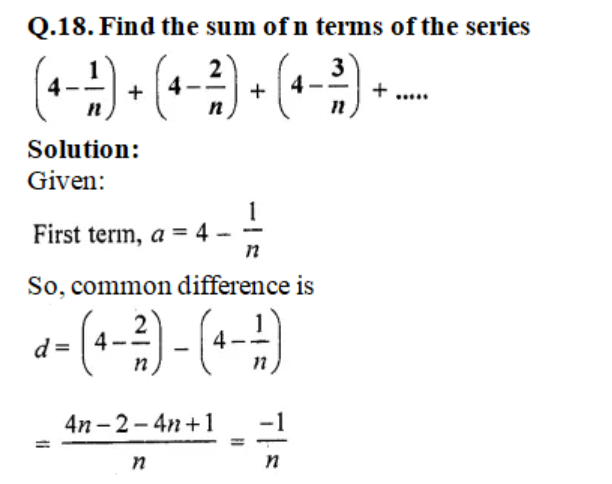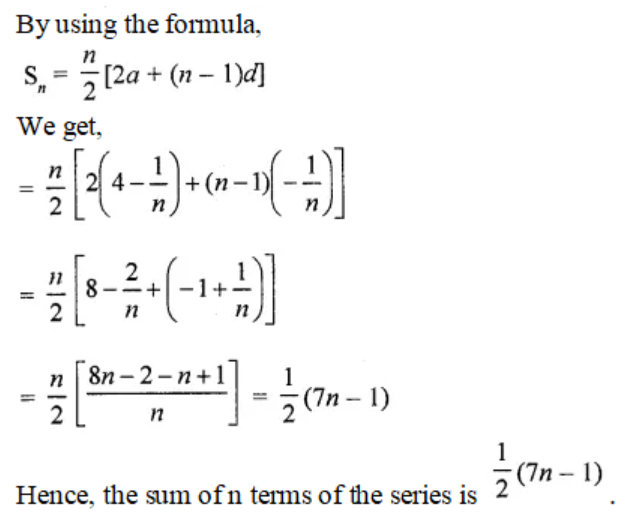19. In an A.P., if the first term is 22, the common difference is – 4 and the sum to n terms is 64, find n.

Solution:

Given that,

a = 22, d = – 4 and Sn = 64

Let us consider the number of terms as n.

For sum of terms in an A.P, we know that

Sn = n/2[2a + (n − 1)d]

Where; a = first term for the given A.P. d = common difference of the given A.P. n = number of terms

So,

⟹ Sn = n/2[2(22) + (n − 1)(−4)]

⟹ 64 = n/2[2(22) + (n − 1)(−4)]

⟹ 64(2) = n(48 – 4n)

⟹ 128 = 48n – 4n2

After rearranging the terms, we have a quadratic equation

4n2 – 48n + 128 = 0,

n2 – 12n + 32 = 0 [dividing by 4 on both sides]

n2 – 12n + 32 = 0

Solving by factorisation method,

n2 – 8n – 4n + 32 = 0

n ( n – 8 ) – 4 ( n – 8 ) = 0

(n – 8) (n – 4) = 0

So, we get n – 8 = 0 ⟹ n = 8

Or, n – 4 = 0 ⟹ n = 4

Hence, the number of terms can be either n = 4 or 8.

20. In an A.P., if the 5th and 12th terms are 30 and 65 respectively, what is the sum of first 20 terms?

Solution:

Let’s take the first term as a and the common difference to be d

Given that,

a5 = 30  and a12 = 65

And, we know that an = a + (n – 1)d

So,

a5 = a + (5 – 1)d

30 = a + 4d

a = 30 – 4d   …. (i)

Similarly, a12 = a + (12 – 1) d

65 = a + 11d

a = 65 – 11d …. (ii)

Subtracting (i) from (ii), we have

a – a = (65 – 11d) – (30 – 4d)

0 = 65 – 11d – 30 + 4d

0 = 35 – 7d

7d = 35

d = 5

Putting d in (i), we get

a = 30 – 4(5)

a = 30 – 20

a = 10

Thus for the A.P; d = 5 and a = 10

Next, to find the sum of first 20 terms of this A.P., we use the following formula for the sum of n terms of an A.P.,

Sn = n/2[2a + (n − 1)d]

Where;

a = first term of the given A.P.

d = common difference of the given A.P.

n = number of terms

Here n = 20, so we have

S20 = 20/2[2(10) + (20 − 1)(5)]

= (10)[20 + (19)(5)]

= (10)[20 + 95]

= (10)

= 1150

Hence, the sum of first 20 terms for the given A.P. is 1150

21. Find the sum of first 51 terms of an A.P. whose second and third terms are 14 and 18 respectively.

Solution:

Let’s take the first term as a and the common difference as d.

Given that,

a2 = 14 and a3 = 18

And, we know that an = a + (n – 1)d

So,

a2 = a + (2 – 1)d

⟹ 14 = a + d

⟹ a = 14 – d …. (i)

Similarly,

a3 = a + (3 – 1)d

⟹ 18 = a + 2d

⟹ a = 18 – 2d …. (ii)

Subtracting (i) from (ii), we have

a – a = (18 – 2d) – (14 – d)

0 = 18 – 2d – 14 + d

0 = 4 – d

d = 4

Putting d in (i), to find a

a = 14 – 4

a = 10

Thus, for the A.P. d = 4 and a = 10

Now, to find sum of terms

Sn = n/2(2a + (n − 1)d)

Where,

a = the first term of the A.P.

d = common difference of the A.P.

n = number of terms So, using the formula for

n = 51,

⟹ S51  = 51/2[2(10) + (51 – 1)(4)]

= 51/2[20 + (40)4]

= 51/2

= 51(110)

= 5610

Hence, the sum of the first 51 terms of the given A.P. is 5610

22. If the sum of 7 terms of an A.P. is 49 and that of 17 terms is 289, find the sum of n terms.

Solution:

Given,

Sum of 7 terms of an A.P. is 49

⟹ S7 = 49

And, sum of 17 terms of an A.P. is 289

⟹ S17 = 289

Let the first term of the A.P be a and common difference as d.

And, we know that the sum of n terms of an A.P is

Sn = n/2[2a + (n − 1)d]

So,

S7 = 49 = 7/2[2a + (7 – 1)d]

= 7/2 [2a + 6d]

= 7[a + 3d]

⟹ 7a + 21d = 49

a + 3d = 7 ….. (i)

Similarly,

S17 = 17/2[2a + (17 – 1)d]

= 17/2 [2a + 16d]

= 17[a + 8d]

⟹ 17[a + 8d] = 289

a + 8d = 17 ….. (ii)

Now, subtracting (i) from (ii), we have

a + 8d – (a + 3d) = 17 – 7

5d = 10

d = 2

Putting d in (i), we find a

a + 3(2) = 7

a = 7 – 6 = 1

So, for the A.P: a = 1 and d = 2

For the sum of n terms is given by,

Sn  = n/2[2(1) + (n − 1)(2)]

= n/2[2 + 2n – 2]

= n/2[2n]

= n2

Therefore, the sum of n terms of the A.P is given by n2.

23. The first term of an A.P. is 5, the last term is 45 and the sum is 400. Find the number of terms and the common difference.

Solution:

Sum of first n terms of an A.P is given by Sn = n/2(2a + (n − 1)d)

Given,

First term (a) = 5, last term (an) = 45 and sum of n terms (Sn) = 400

Now, we know that

an = a + (n – 1)d

⟹ 45 = 5 + (n – 1)d

⟹ 40 = nd – d

⟹ nd – d = 40 …. (1)

Also,

S= n/2(2(a) + (n − 1)d)

400 = n/2(2(5) + (n − 1)d)

800 = n (10 + nd – d)

800 = n (10 + 40) [using (1)]

⟹ n = 16

Putting n in (1), we find d

nd – d = 40

16d – d = 40

15d = 40

d = 8/3

Therefore, the common difference of the given A.P. is 8/3.

24. In an A.P. the first term is 8, nth term is 33 and the sum of first n term is 123. Find n and the d, the common difference.

Solution:

Given,

The first term of the A.P (a) = 8

The nth term of the A.P (l) = 33

And, the sum of all the terms Sn = 123

Let the common difference of the A.P. be d.

So, find the number of terms by

123 = (n/2)(8 + 33)

123 = (n/2)(41)

n = (123 x 2)/ 41

n = 246/41

n = 6

Next, to find the common difference of the A.P. we know that

l = a + (n – 1)d

33 = 8 + (6 – 1)d

33 = 8 + 5d

5d = 25

d = 5

Thus, the number of terms is n = 6 and the common difference of the A.P. is d = 5.

25. In an A.P. the first term is 22, nth term is -11 and the sum of first n term is 66. Find n and the d, the common difference.

Solution:

Given,

The first term of the A.P (a) = 22

The nth term of the A.P (l) = -11

And, sum of all the terms Sn = 66

Let the common difference of the A.P. be d.

So, finding the number of terms by

66 = (n/2)[22 + (−11)]

66 = (n/2)[22 − 11]

(66)(2) = n(11)

6 × 2 = n

n = 12

Now, for finding d

We know that, l = a + (n – 1)d

– 11 = 22 + (12 – 1)d

-11 = 22 + 11d

11d = – 33

d = – 3

Hence, the number of terms is n = 12 and the common difference d = -3

26. The first and the last terms of an A.P. are 7 and 49 respectively. If sum of all its terms is 420, find the common difference.

Solution:

Given,

First term (a) = 7, last term (an) = 49 and sum of n terms (Sn) = 420

Now, we know that

an = a + (n – 1)d

⟹ 49 = 7 + (n – 1)d

⟹ 43 = nd – d

⟹ nd – d = 42  ….. (1)

Next,

Sn = n/2(2(7) + (n − 1)d)

⟹ 840 = n[14 + nd – d]

⟹ 840 = n[14 + 42] [using (1)]

⟹ 840 = 54n

⟹ n = 15 …. (2)

So, by substituting (2) in (1), we have

nd – d = 42

⟹ 15d – d = 42

⟹ 14d = 42

⟹ d = 3

Therefore, the common difference of the given A.P. is 3.

27. The first and the last terms of an A.P are 5 and 45 respectively. If the sum of all its terms is 400, find its common difference.

Solution:

Given,

First term (a) = 5 and the last term (l) = 45

Also, Sn = 400

We know that,

an = a + (n – 1)d

⟹ 45 = 5 + (n – 1)d

⟹ 40 = nd – d

⟹ nd – d = 40  ….. (1)

Next,

Sn = n/2(2(5) + (n − 1)d)

⟹ 400 = n[10 + nd – d]

⟹ 800 = n[10 + 40] [using (1)]

⟹ 800 = 50n

⟹ n = 16 …. (2)

So, by substituting (2) in (1), we have

nd – d = 40

⟹ 16d – d = 40

⟹ 15d = 40

⟹ d = 8/3

Therefore, the common difference of the given A.P. is 8/3.

28. The sum of first q terms of an A.P. is 162. The ratio of its 6th term to its 13th term is 1: 2. Find the first and 15th term of the A.P.

Solution:

Let a be the first term and d be the common difference.

And we know that, sum of first n terms is:

Sn = n/2(2a + (n − 1)d)

Also, nth term is given by an = a + (n – 1)d

From the question, we have

Sq = 162 and a: a13 = 1 : 2

So,

2a= a13

⟹ 2 [a + (6 – 1d)] = a + (13 – 1)d

⟹ 2a + 10d = a + 12d

⟹ a = 2d  …. (1)

And, S9 = 162

⟹ S9 = 9/2(2a + (9 − 1)d)

⟹ 162 = 9/2(2a + 8d)

⟹ 162 × 2 = 9[4d + 8d]  [from (1)]

⟹ 324 = 9 × 12d

⟹ d = 3

⟹ a = 2(3) [from (1)]

⟹ a = 6

Hence, the first term of the A.P. is 6

For the 15th term, a15 = a + 14d = 6 + 14 × 3 = 6 + 42

Therefore, a15 = 48

29. If the 10th term of an A.P. is 21 and the sum of its first 10 terms is 120, find its nth term.

Solution:

Let’s consider a to be the first term and d be the common difference.

And we know that, sum of first n terms is:

Sn = n/2(2a + (n − 1)d) and nth term is given by: an = a + (n – 1)d

Now, from the question we have

S10 = 120

⟹ 120 = 10/2(2a + (10 − 1)d)

⟹ 120 = 5(2a + 9d)

⟹ 24 = 2a + 9d …. (1)

Also given that, a10 = 21

⟹ 21 = a + (10 – 1)d

⟹ 21 = a + 9d …. (2)

Subtracting (2) from (1), we get

24 – 21 = 2a + 9d – a – 9d

⟹a = 3

Now, on putting a = 3 in equation (2), we get

3 + 9d = 21

9d = 18

d = 2

Thus, we have the first term(a) = 3 and the common difference(d) = 2

Therefore, the nth term is given by

an  = a + (n – 1)d = 3 + (n – 1)2

= 3 + 2n -2

= 2n + 1

Hence, the nth term of the A.P is (an) = 2n + 1.

30. The sum of first 7 terms of an A.P. is 63 and the sum of its next 7 terms is 161. Find the 28th term of this A.P.

Solution:

Let’s take a to be the first term and d to be the common difference.

And we know that, sum of first n terms

Sn = n/2(2a + (n − 1)d)

Given that sum of the first 7 terms of an A.P. is 63.

S7 = 63

And sum of next 7 terms is 161.

So, the sum of first 14 terms = Sum of first 7 terms + sum of next 7 terms

S14 = 63 + 161 = 224

Now, having

S7 = 7/2(2a + (7 − 1)d)

⟹ 63(2) = 7(2a + 6d)

⟹ 9 × 2 = 2a + 6d

⟹ 2a + 6d = 18 . . . . (1)

And,

S14 = 14/2(2a + (14 − 1)d)

⟹ 224 = 7(2a + 13d)

⟹ 32 = 2a + 13d …. (2)

Now, subtracting (1) from (2), we get

⟹ 13d – 6d = 32 – 18

⟹ 7d = 14

⟹ d = 2

Using d in (1), we have

2a + 6(2) = 18

2a = 18 – 12

a = 3

Thus, from nth term

⟹ a28  = a + (28 – 1)d

= 3 + 27 (2)

= 3 + 54 = 57

Therefore, the 28th term is 57.

31. The sum of first seven terms of an A.P. is 182. If its 4th and 17th terms are in ratio 1: 5, find the A.P.

Solution:

Given that,

S17 = 182

And, we know that the sum of first n term is:

S= n/2(2a + (n − 1)d)

So,

S7 = 7/2(2a + (7 − 1)d)

182 × 2 = 7(2a + 6d)

364 = 14a + 42d

26 = a + 3d

a = 26 – 3d … (1)

Also, it’s given that 4th term and 17th term are in a ratio of 1: 5. So, we have

⟹ 5(a4) = 1(a17)

⟹ 5 (a + 3d) = 1 (a + 16d)

⟹ 5a + 15d = a + 16d

⟹ 4a = d …. (2)

Now, substituting (2) in (1), we get

⟹ 4 ( 26 – 3d ) = d

⟹ 104 – 12d = d

⟹ 104 = 13d

⟹ d = 8

Putting d in (2), we get

⟹ 4a = d

⟹ 4a = 8

⟹ a = 2

Therefore, the first term is 2 and the common difference is 8. So, the A.P. is 2, 10, 18, 26, . ..

32. The nth term of an A.P is given by (-4n + 15). Find the sum of first 20 terms of this A.P.

Solution:

Given,

The nth term of the A.P = (-4n + 15)

So, by putting n = 1 and n = 20 we can find the first ans 20th term of the A.P

a = (-4(1) + 15) = 11

And,

a20 = (-4(20) + 15) = -65

Now, for find the sum of 20 terms of this A.P we have the first and last term.

So, using the formula

Sn = n/2(a + l)

S20  = 20/2(11 + (-65))

= 10(-54)

= -540

Therefore, the sum of first 20 terms of this A.P. is -540.

33. In an A.P. the sum of first ten terms is -150 and the sum of its next 10 term is -550. Find the A.P.

Solution:

Let’s take a to be the first term and d to be the common difference.

And we know that, sum of first n terms

Sn = n/2(2a + (n − 1)d)

Given that sum of the first 10 terms of an A.P. is -150.

S10 = -150

And the sum of next 10 terms is -550.

So, the sum of first 20 terms = Sum of first 10 terms + sum of next 10 terms

S20 = -150 + -550 = -700

Now, having

S10 = 10/2(2a + (10 − 1)d)

⟹ -150 = 5(2a + 9d)

⟹ -30 = 2a + 9d

⟹ 2a + 9d = -30 . . . . (1)

And,

S20 = 20/2(2a + (20 − 1)d)

⟹ -700 = 10(2a + 19d)

⟹ -70 = 2a + 19d …. (2)

Now, subtracting (1) from (2), we get

⟹ 19d – 9d = -70 – (-30)

⟹ 10d = -40

⟹ d = -4

Using d in (1), we have

2a + 9(-4) = -30

2a = -30 + 36

a = 6/2 = 3

Hence, we have a = 3 and d = -4

So, the A.P is 3, -1, -5, -9, -13,…..

34. Sum of the first 14 terms of an A.P. is 1505 and its first term is 10. Find its 25th term.

Solution

Given,

First term of the A.P is 1505 and

S14 = 1505

We know that, the sum of first n terms is

Sn = n/2(2a + (n − 1)d)

So,

S14 = 14/2(2(10) + (14 − 1)d) = 1505

7(20 + 13d) = 1505

20 + 13d = 215

13d = 215 – 20

d = 195/13

d =15

Thus, the 25th term is given by

a25 = 10 + (25 -1)15

= 10 + (24)15

= 10 + 360

= 370

Therefore, the 25th term of the A.P is 370

35. In an A.P. , the first term is 2, the last term is 29 and the sum of the terms is 155. Find the common difference of the A.P.

Solution:

Given,

The first term of the A.P. (a) = 2

The last term of the A.P. (l) = 29

And, sum of all the terms (Sn) = 155

Let the common difference of the A.P. be d.

So, find the number of terms by sum of terms formula

Sn = n/2 (a + l)

155 = n/2(2 + 29)

155(2) = n(31)

31n = 310

n = 10

Using n for the last term, we have

l = a + (n – 1)d

29 = 2 + (10 – 1)d

29 = 2 + (9)d

29 – 2 = 9d

9d = 27

d = 3

Hence, the common difference of the A.P. is d = 3

36. The first and the last term of an A.P are 17 and 350 respectively. If the common difference is 9, how many terms are there and what is their sum?

Solution:

Given,

In an A.P first term (a) = 17 and the last term (l) = 350

And, the common difference (d) = 9

We know that,

an = a + (n – 1)d

so,

an = l = 17 + (n – 1)9 = 350

17 + 9n – 9 = 350

9n = 350 – 8

n = 342/9

n = 38

So, the sum of all the term of the A.P is given by

Sn = n/2 (a + l)

= 38/2(17 + 350)

= 19(367)

= 6973

Therefore, the sum of terms of the A.P is 6973.

37. Find the number of terms of the A.P. –12, –9, –6, . . . , 21. If 1 is added to each term of this A.P., then find the sum of all terms of the A.P. thus obtained.

Solution:

Given,

First term, a = -12

Common difference, d = a2 – a1 = – 9 – (- 12)

d = – 9 + 12 = 3

And, we know that nth term = an = a + (n – 1)d

⟹ 21 = -12 + (n – 1)3

⟹ 21 = -12 + 3n – 3

⟹ 21 = 3n – 15

⟹ 36 = 3n

⟹  n = 12

Thus, the number of terms is 12.

Now, if 1 is added to each of the 12 terms, the sum will increase by 12.

Hence, the sum of all the terms of the A.P. so obtained is

⟹ S12 + 12  = 12/2[a + l] + 12

= 6[-12 + 21] + 12

= 6 × 9 + 12

= 66

Therefore, the sum after adding 1 to each of the terms in the A.P is 66.